# Concise Selina Solutions for Class 9 Maths Chapter 7-Indices [Exponents]

Selina Solutions are useful for students as it helps them in scoring high marks in the examination. The Selina Solutions contain detailed step-by-step explanation of all the problems that come under the Chapter 7, Indices [Exponents], of the Class 9 Selina Textbook.

These solutions are prepared by subject matter experts at BYJU’S, describing the complete method of solving problems. By understanding the concepts used in Selina Solutions for Class 9 Maths, students will be able to clear all their doubts related to “Indices [Exponents]”.

### Download PDF of Selina Solutions for Class 9 Maths Chapter 7:-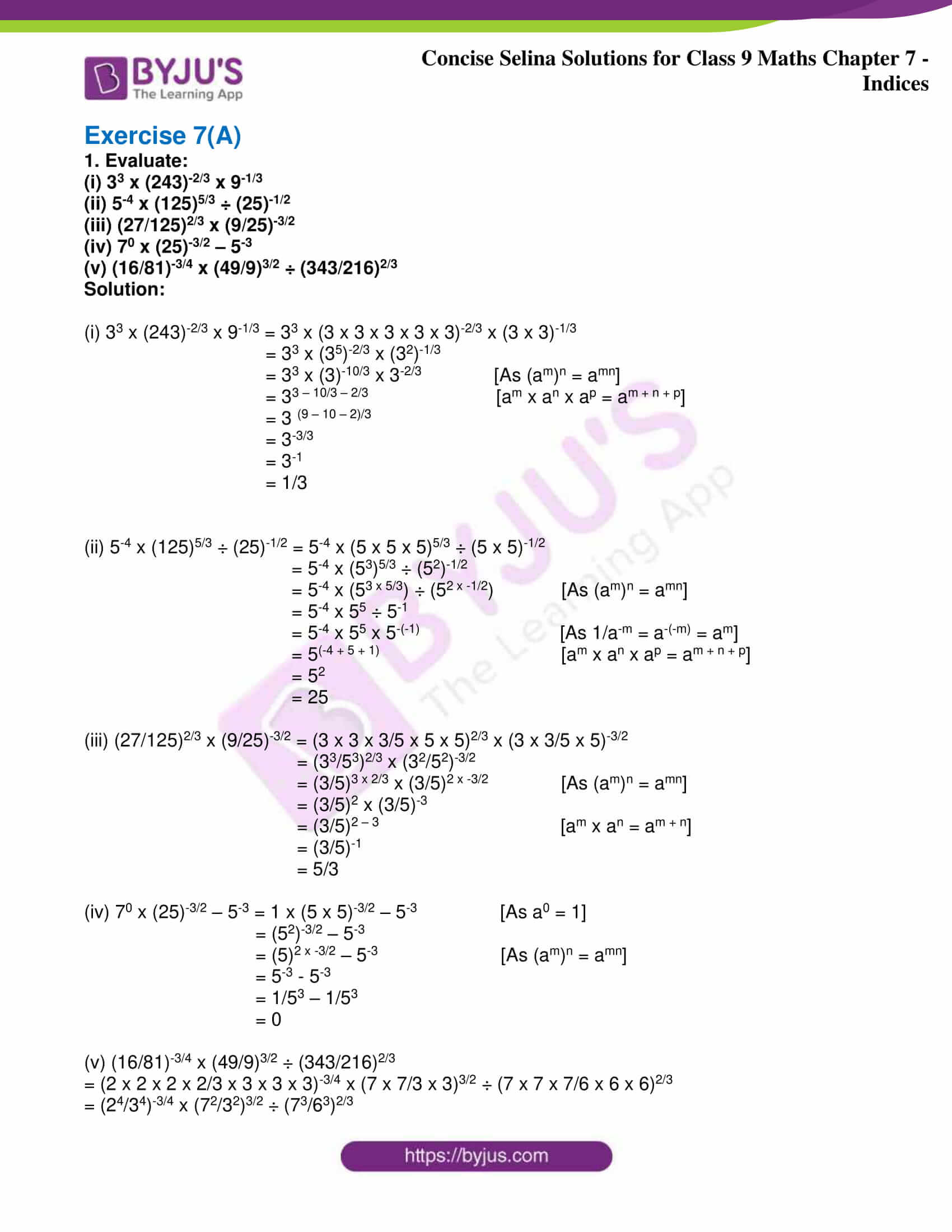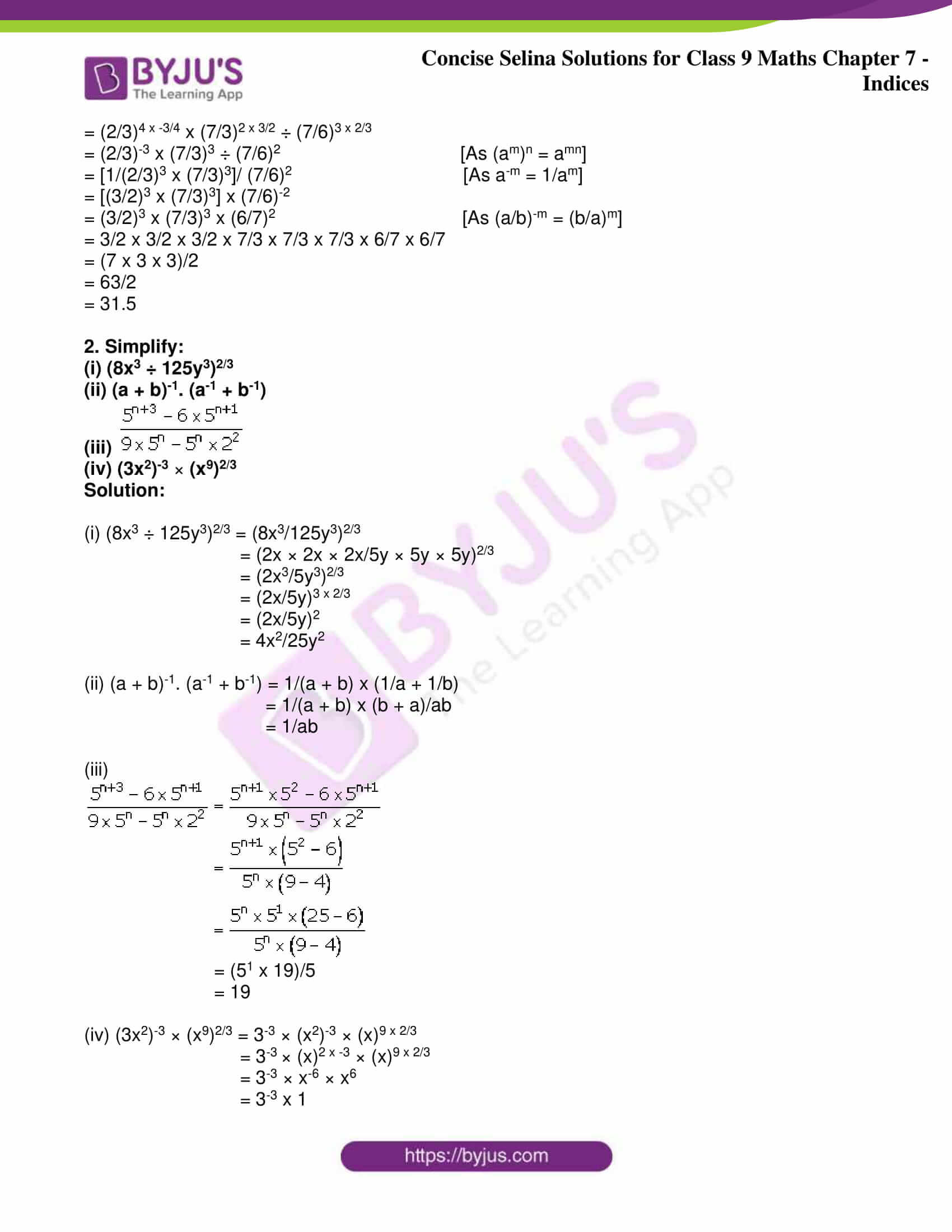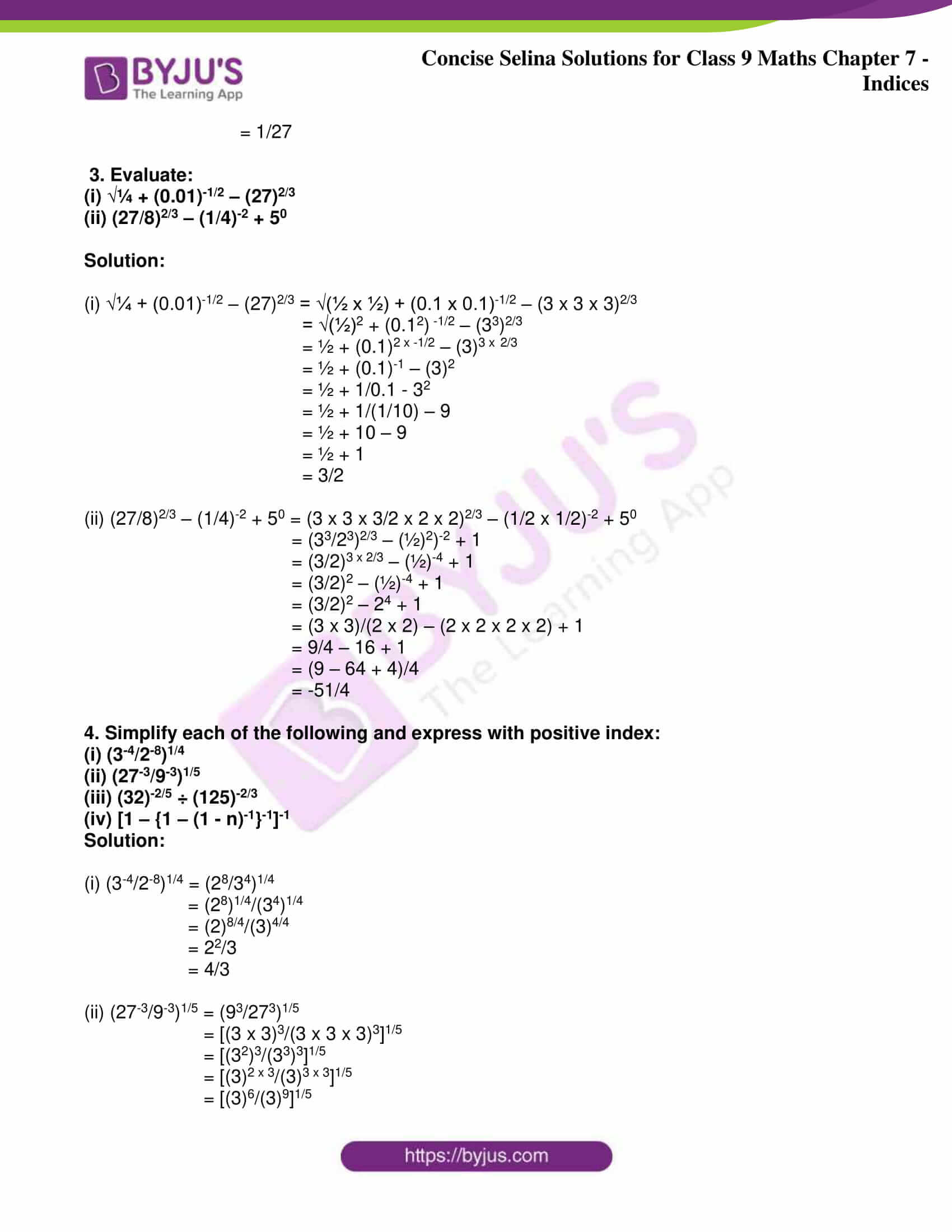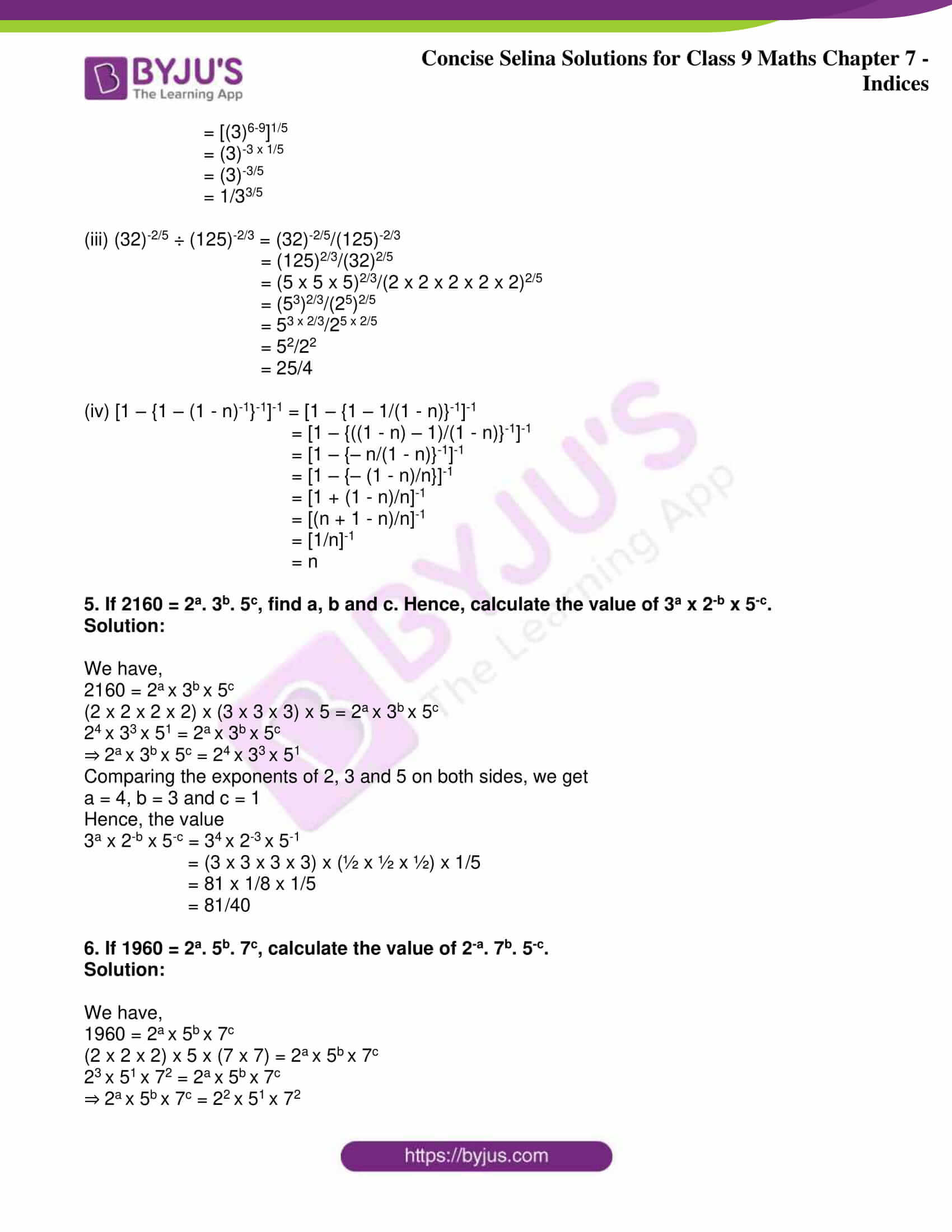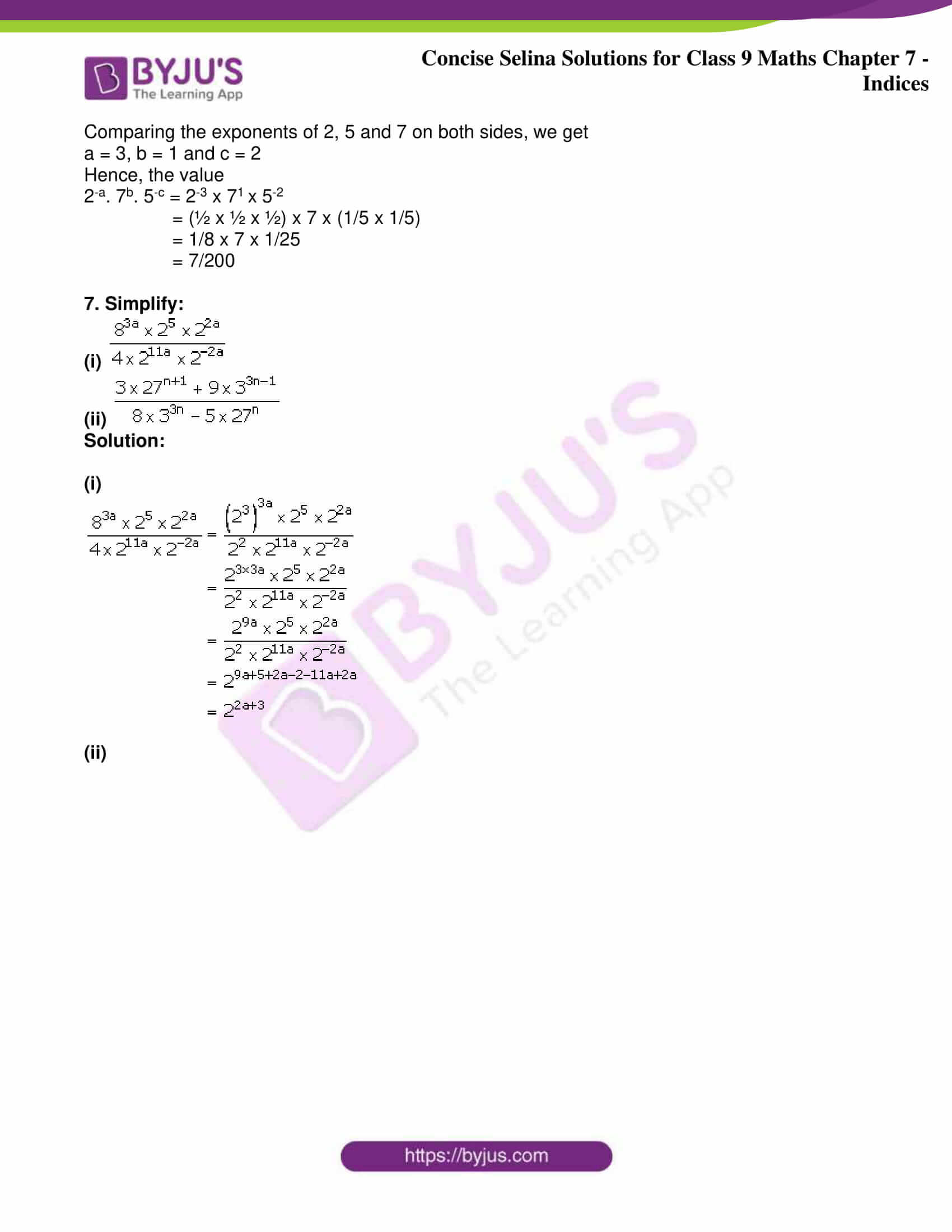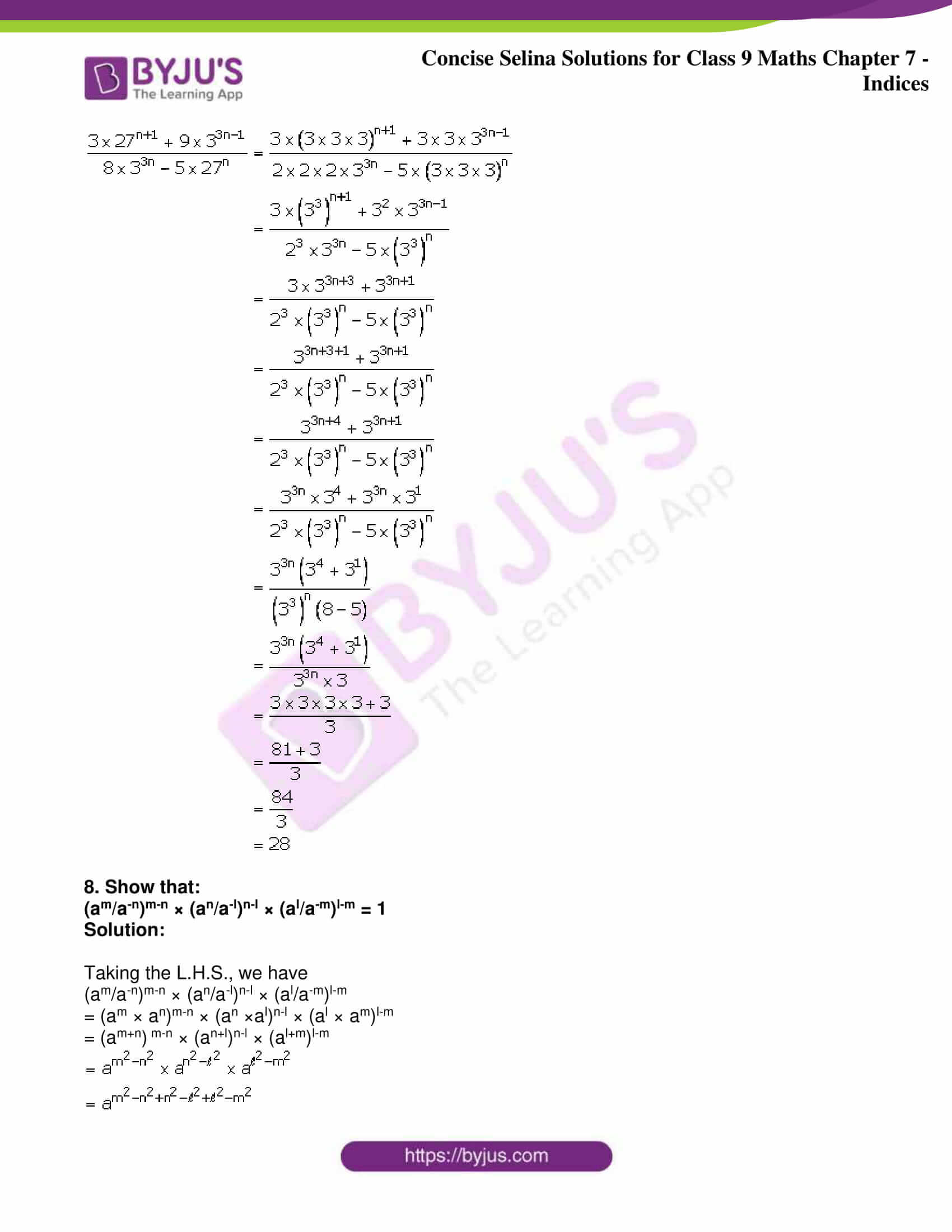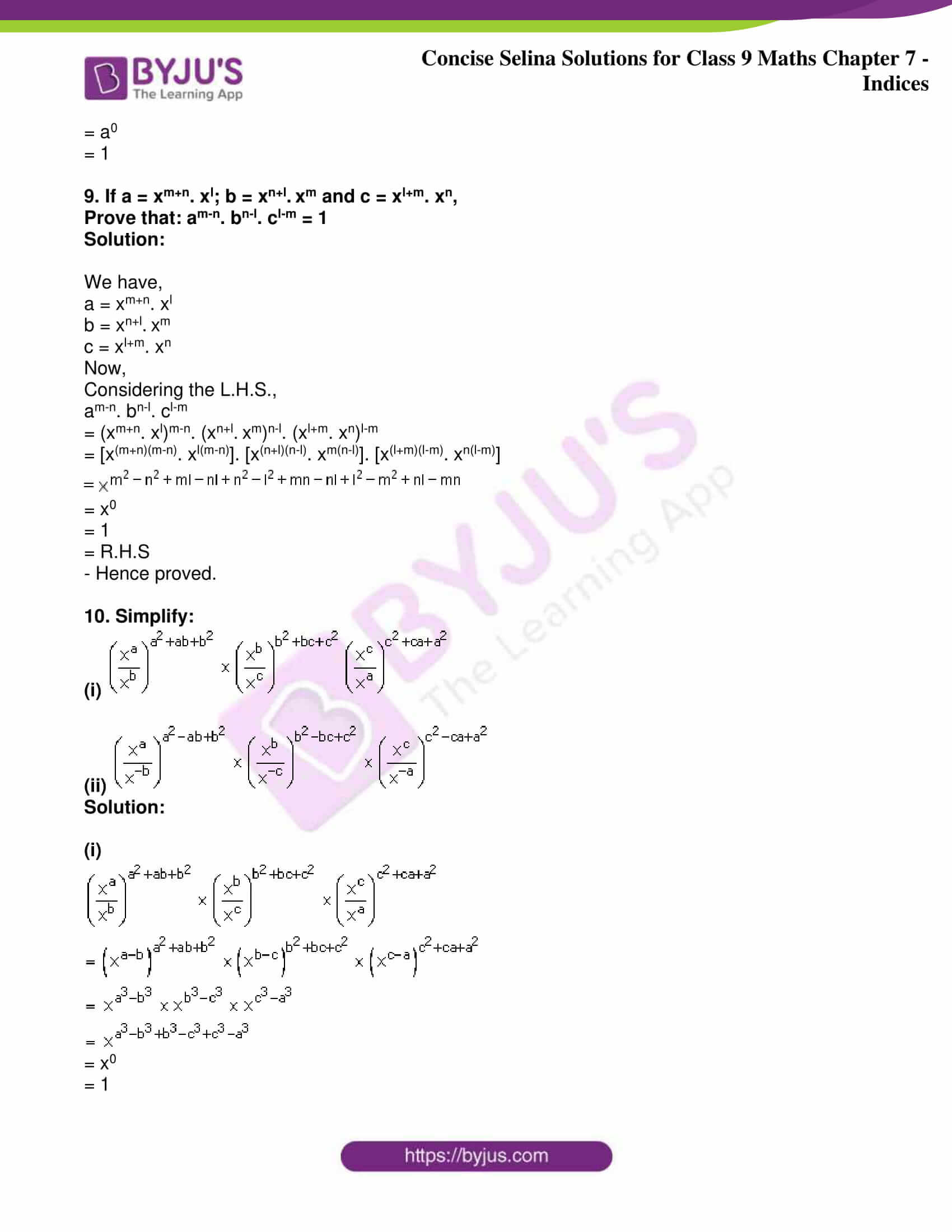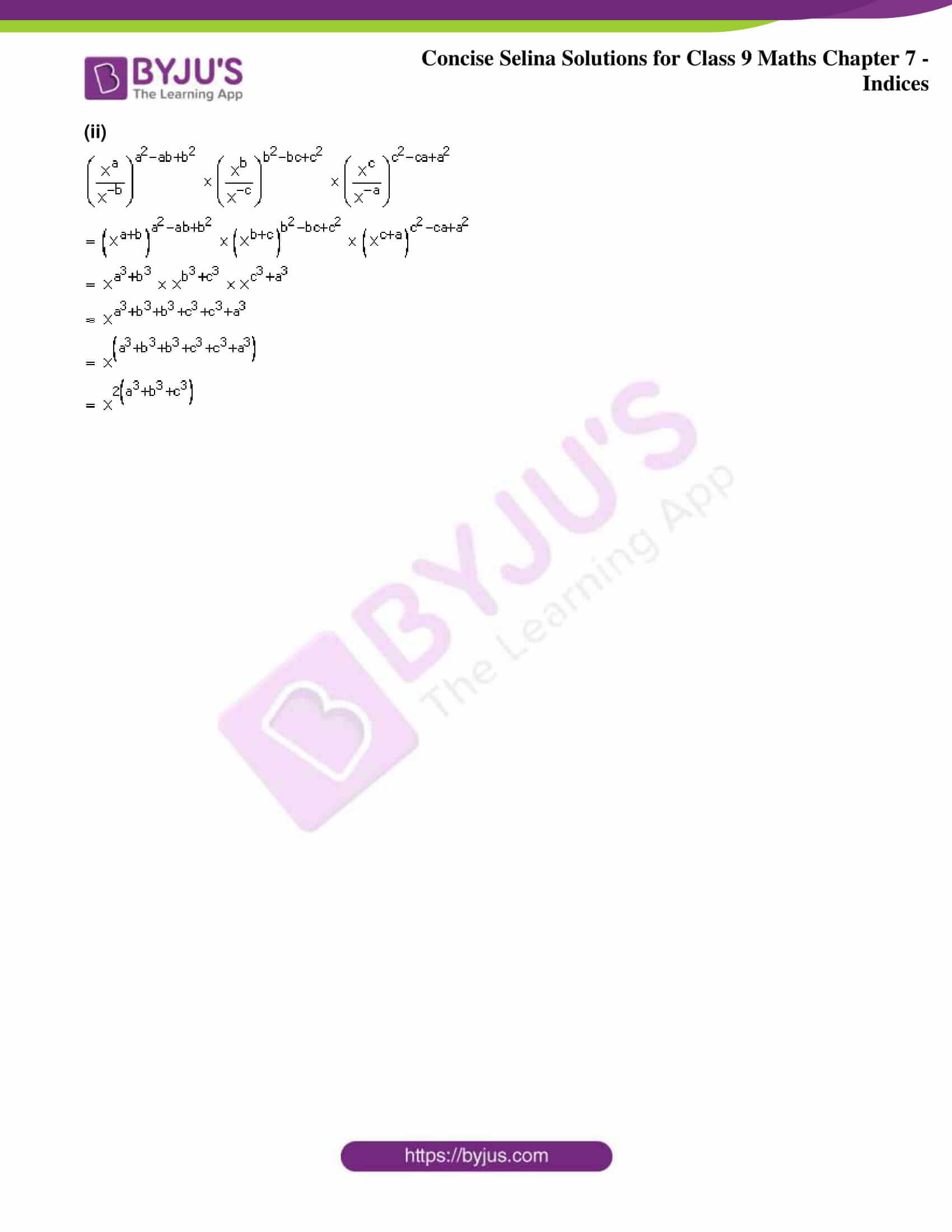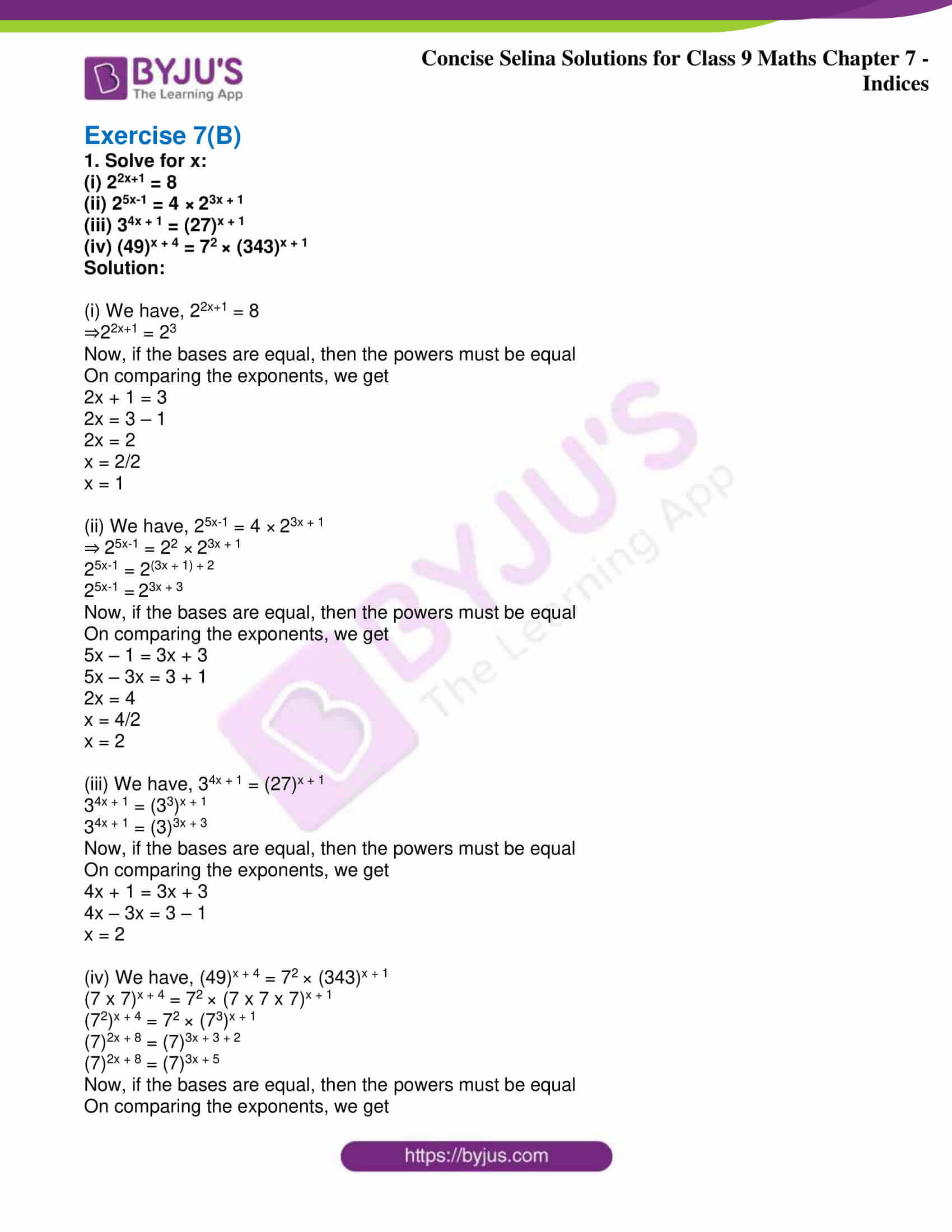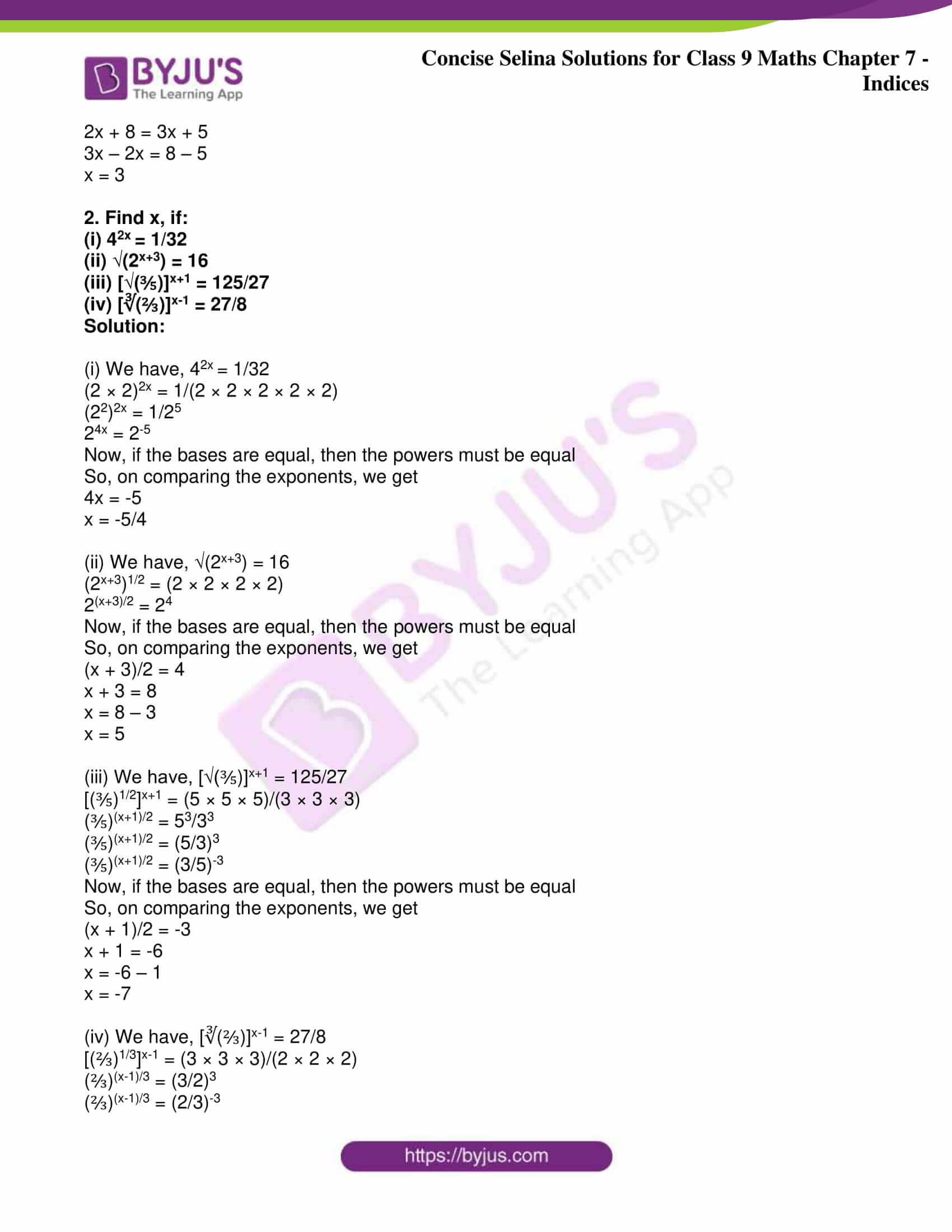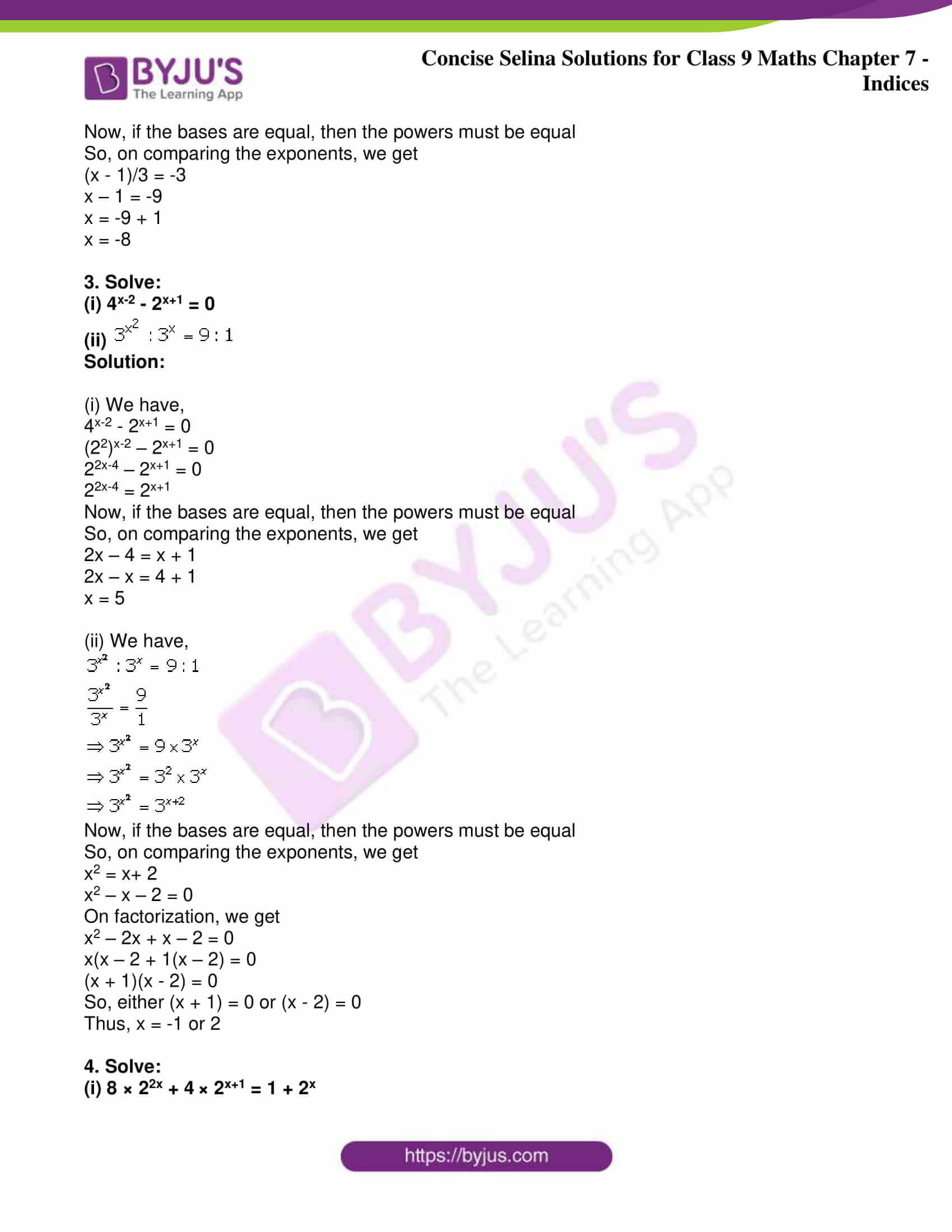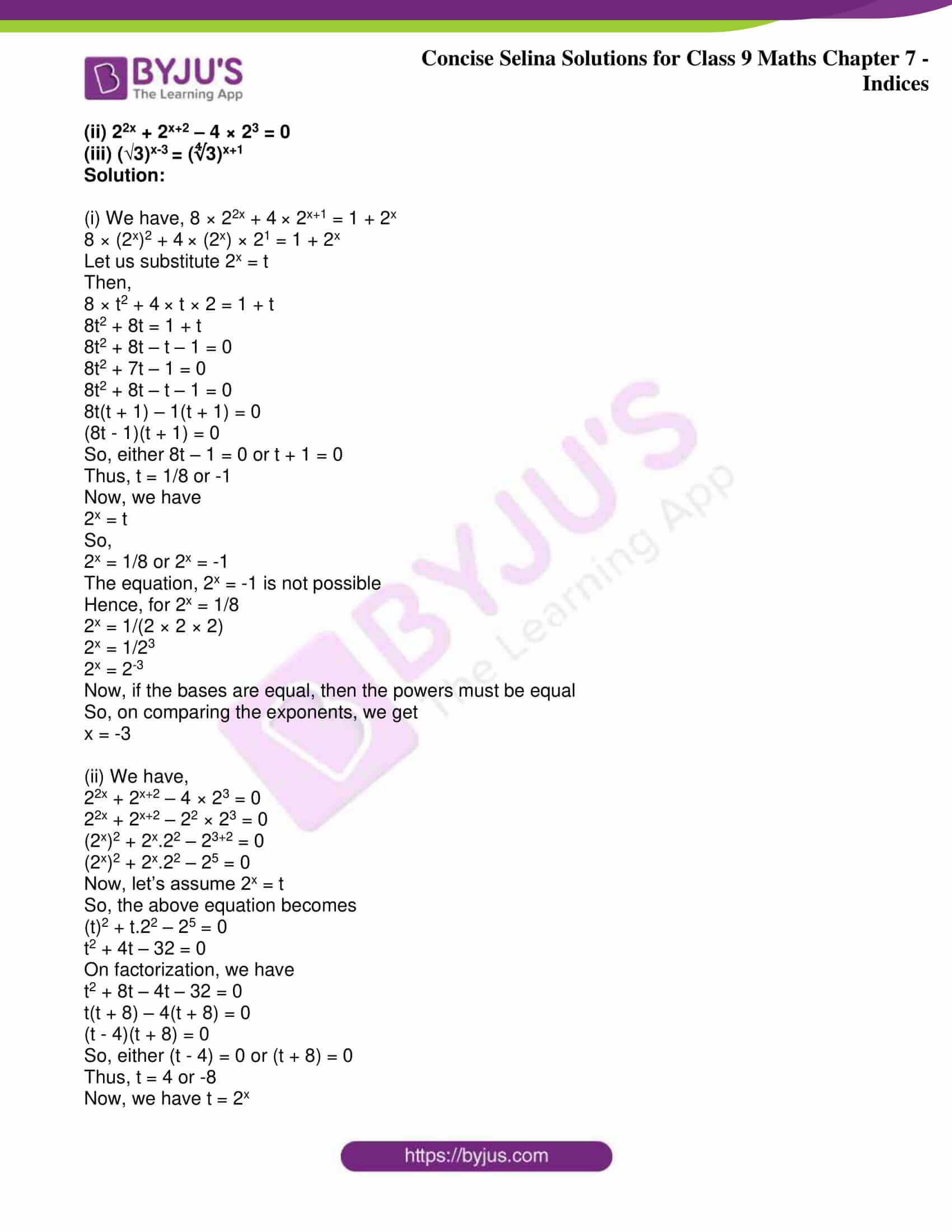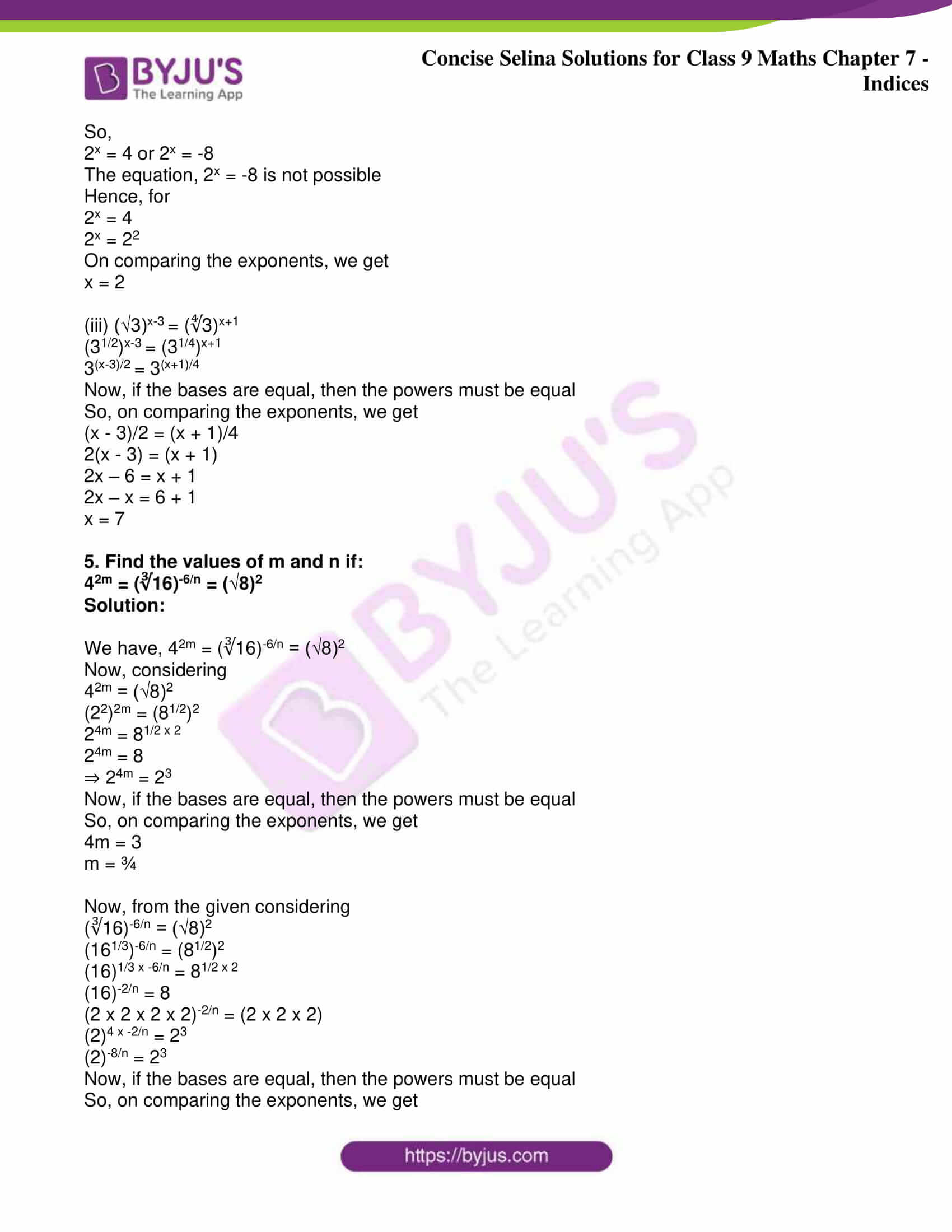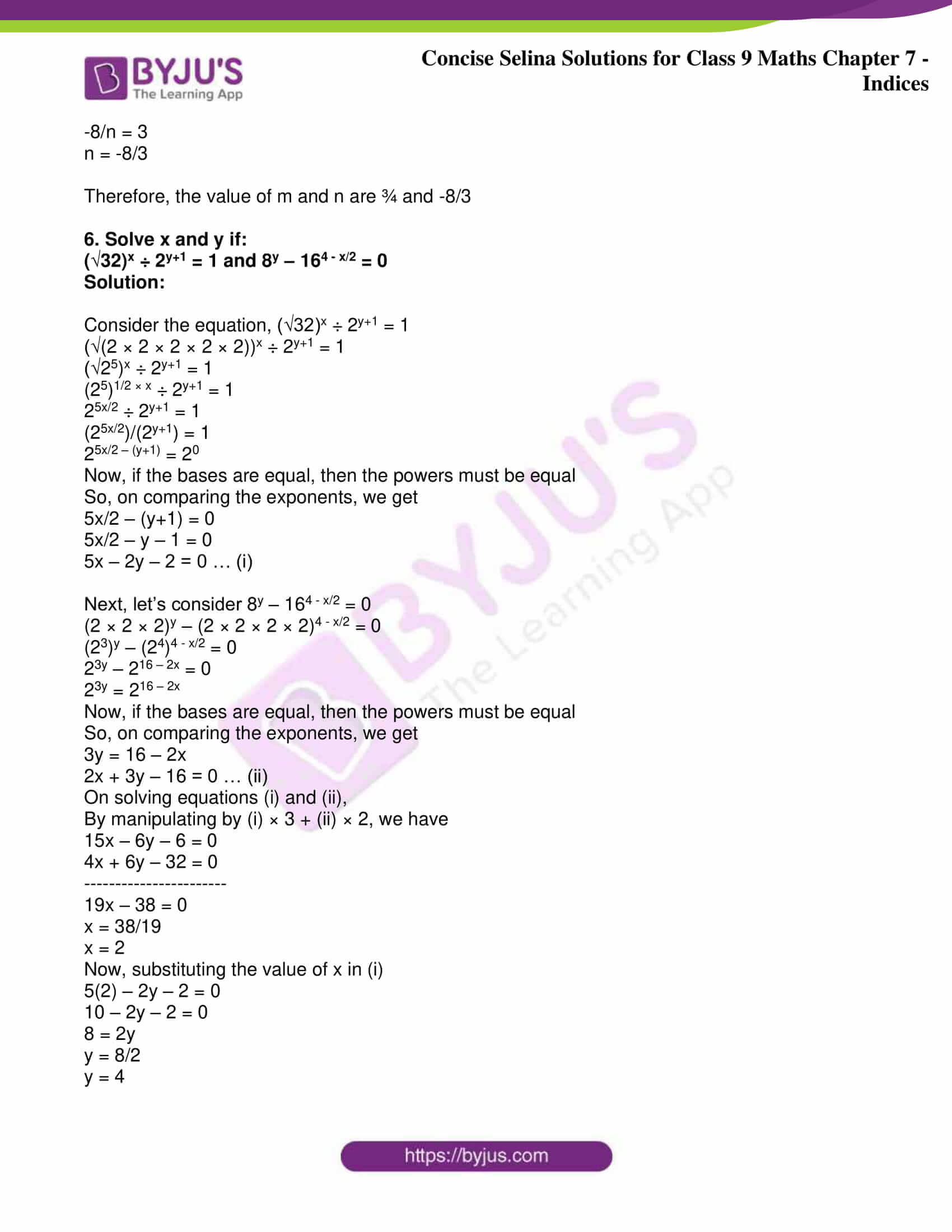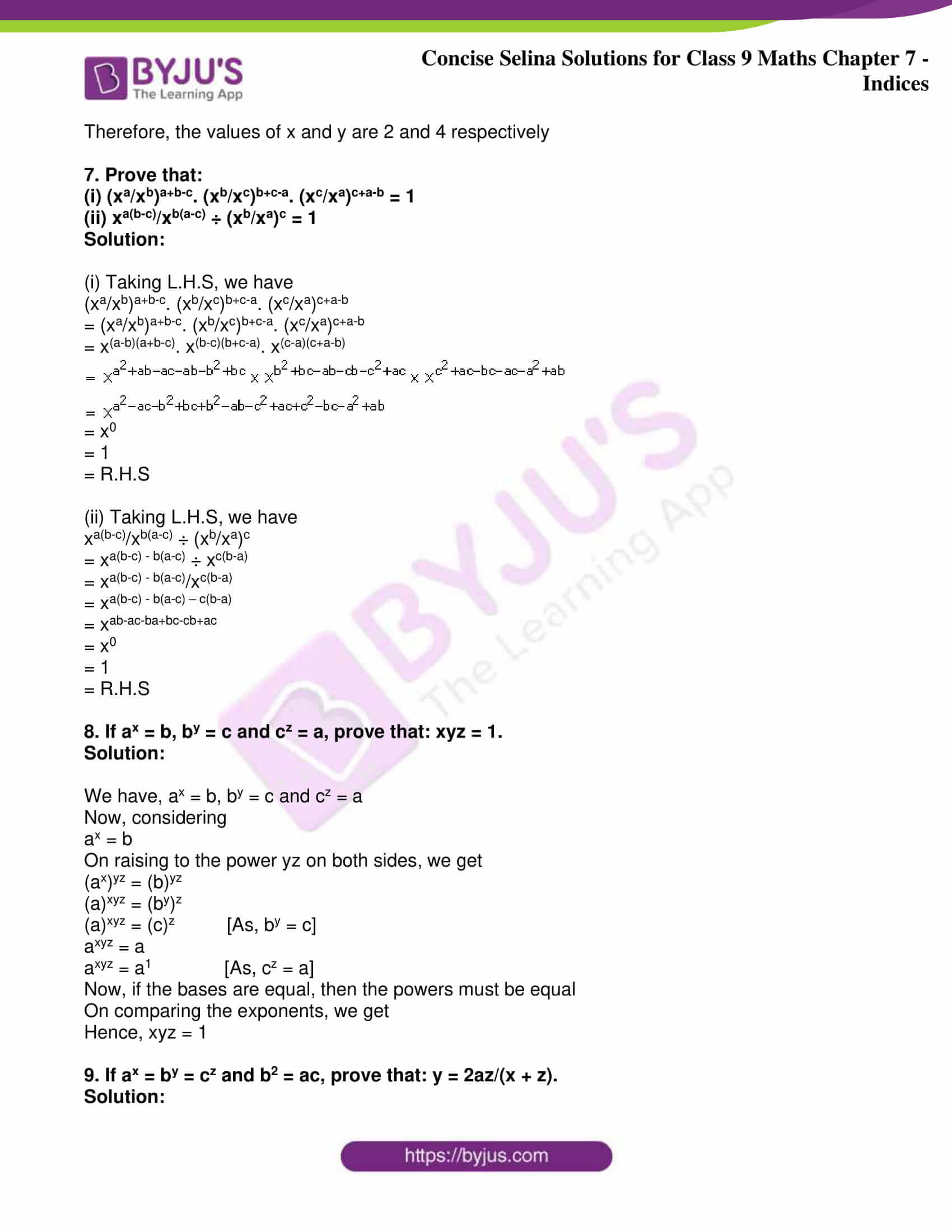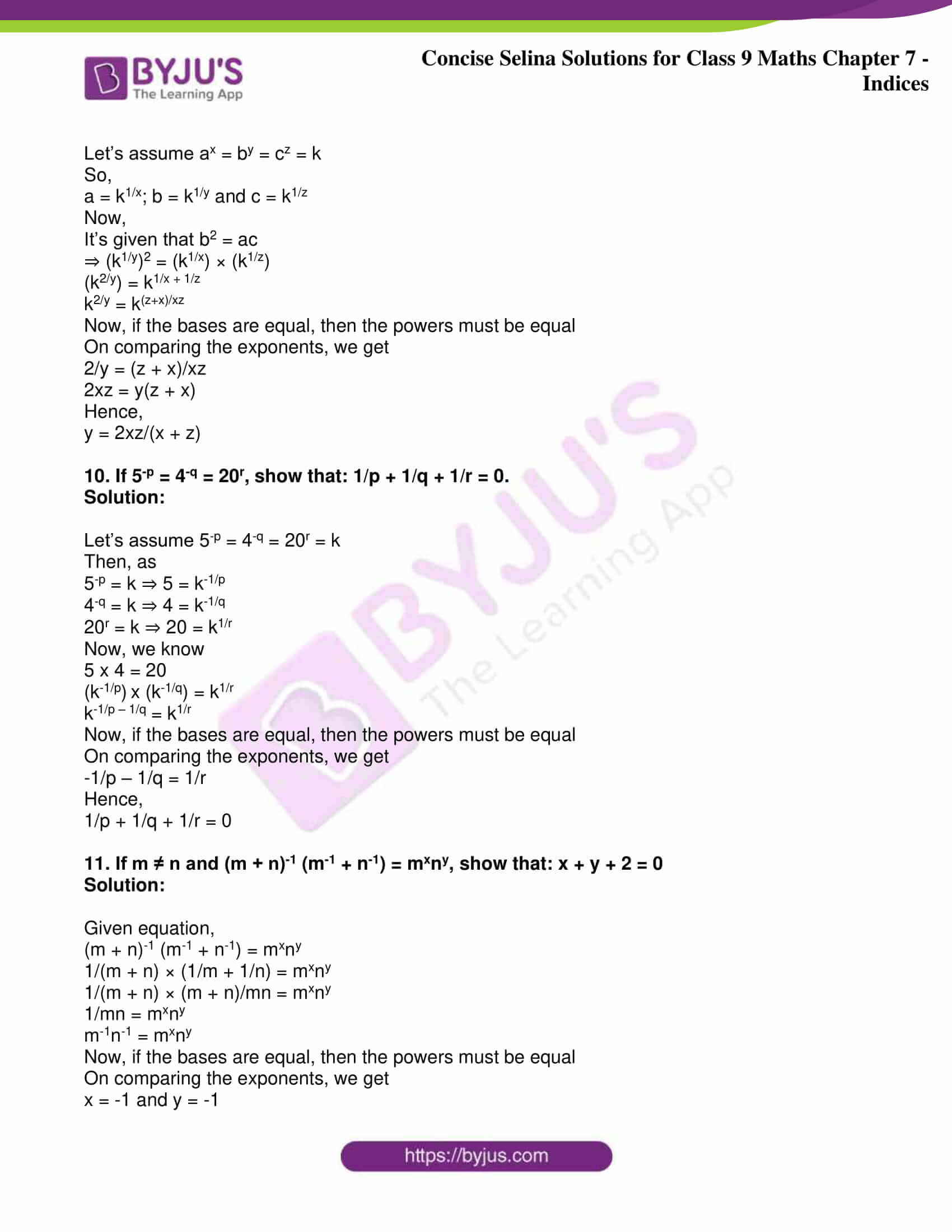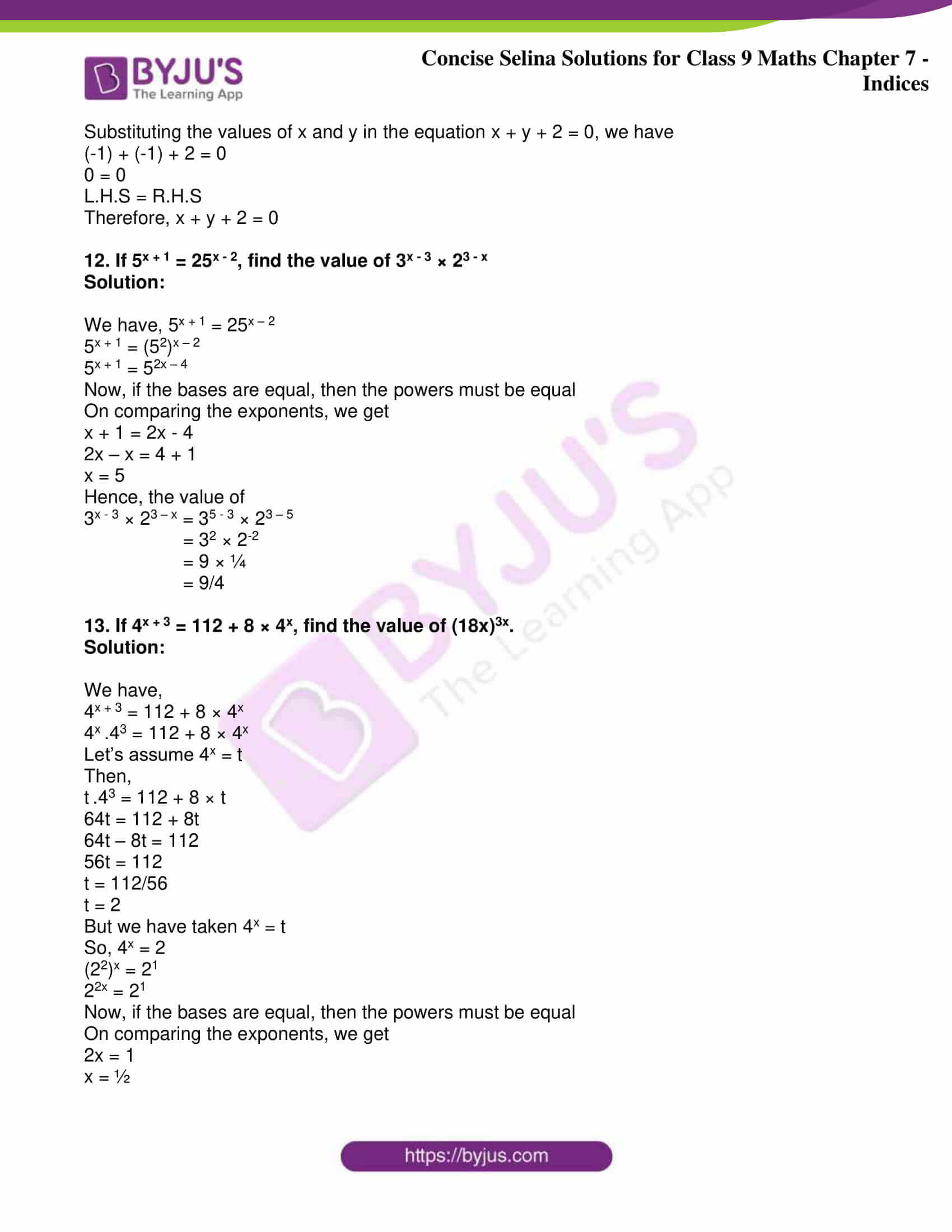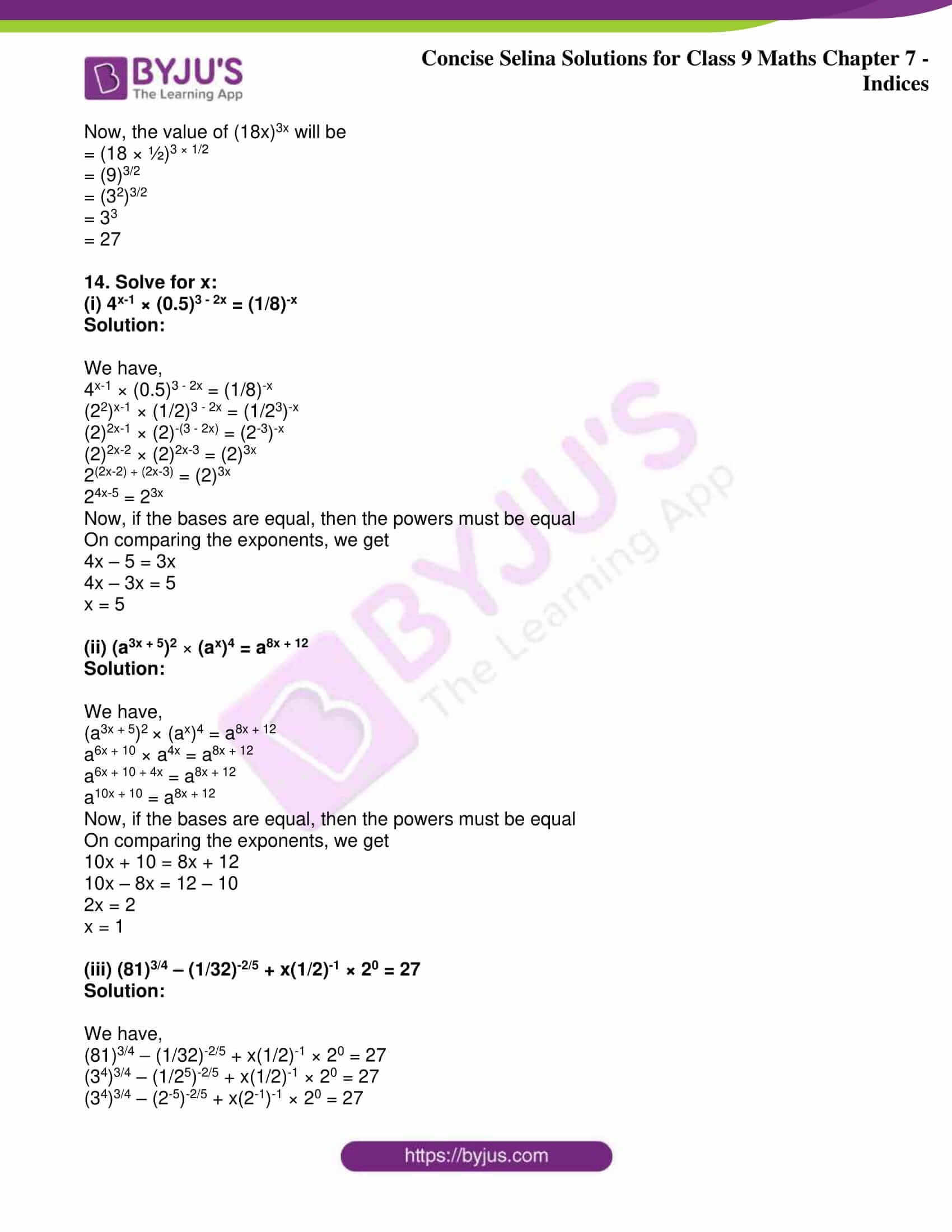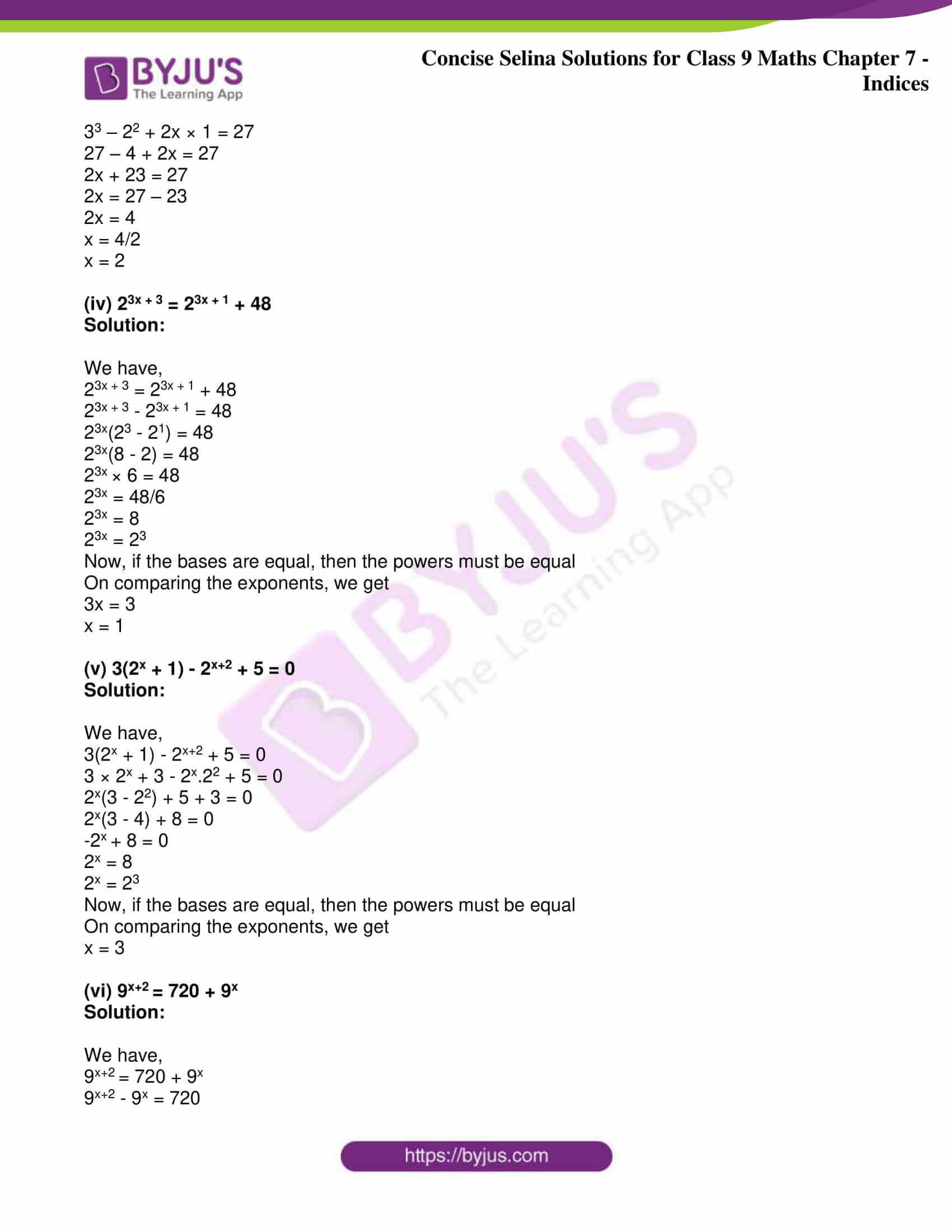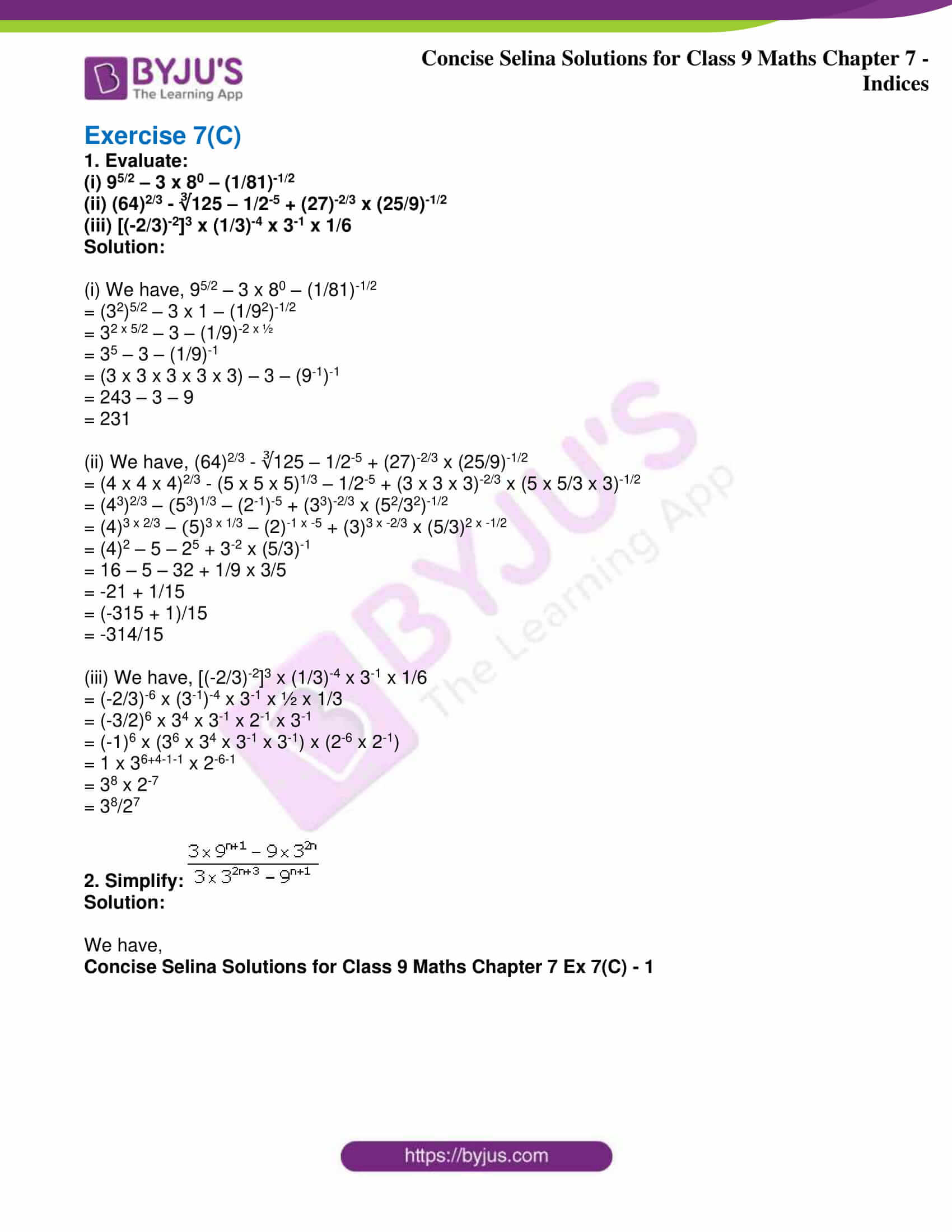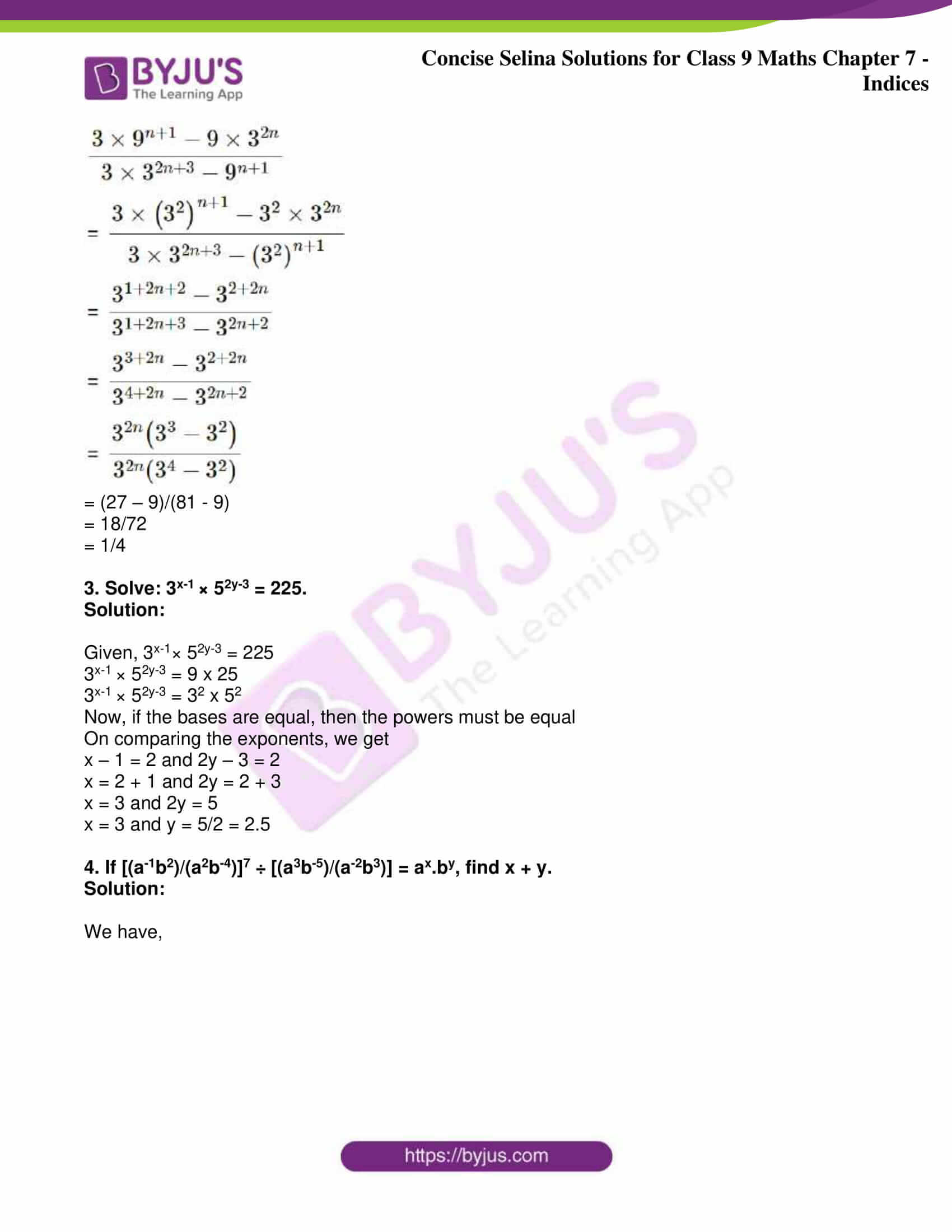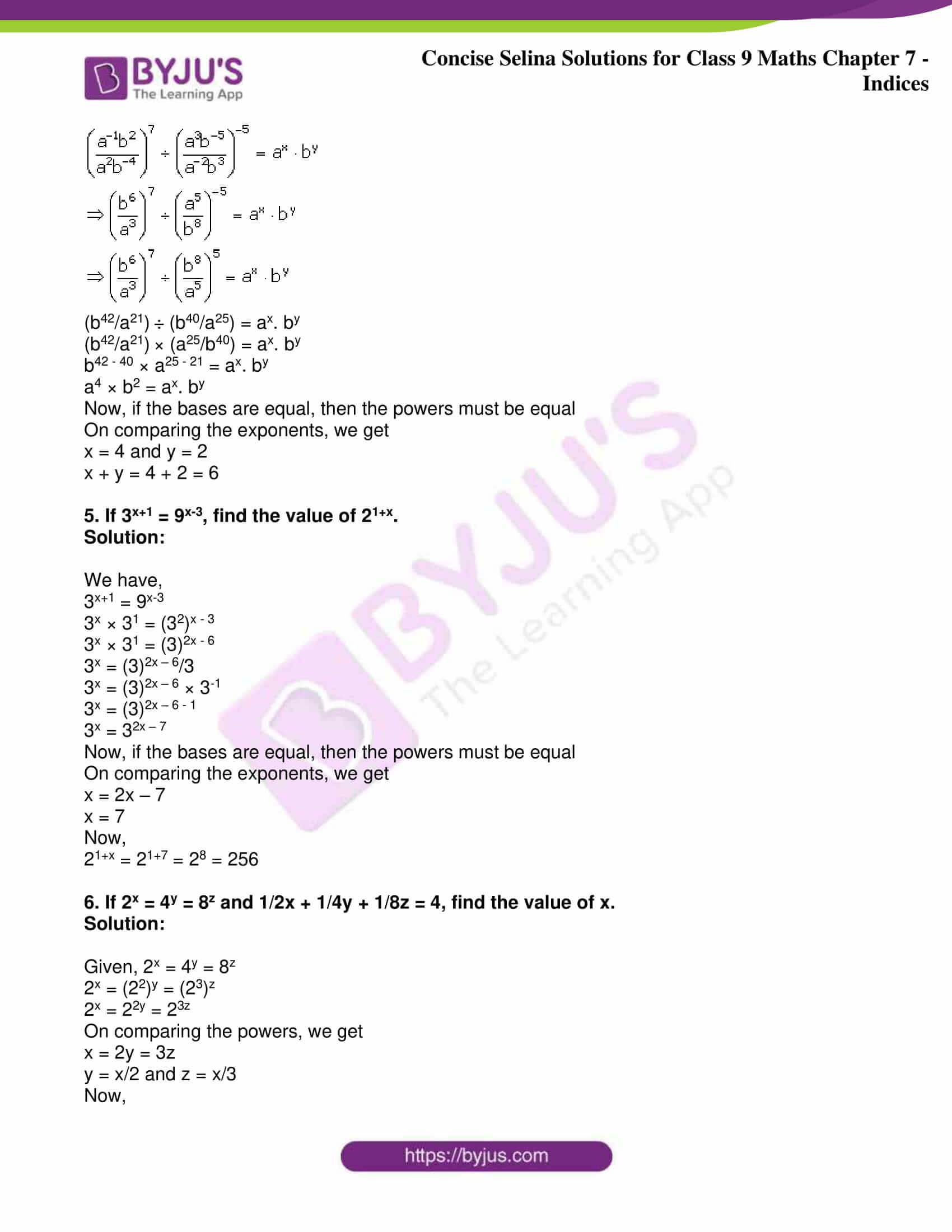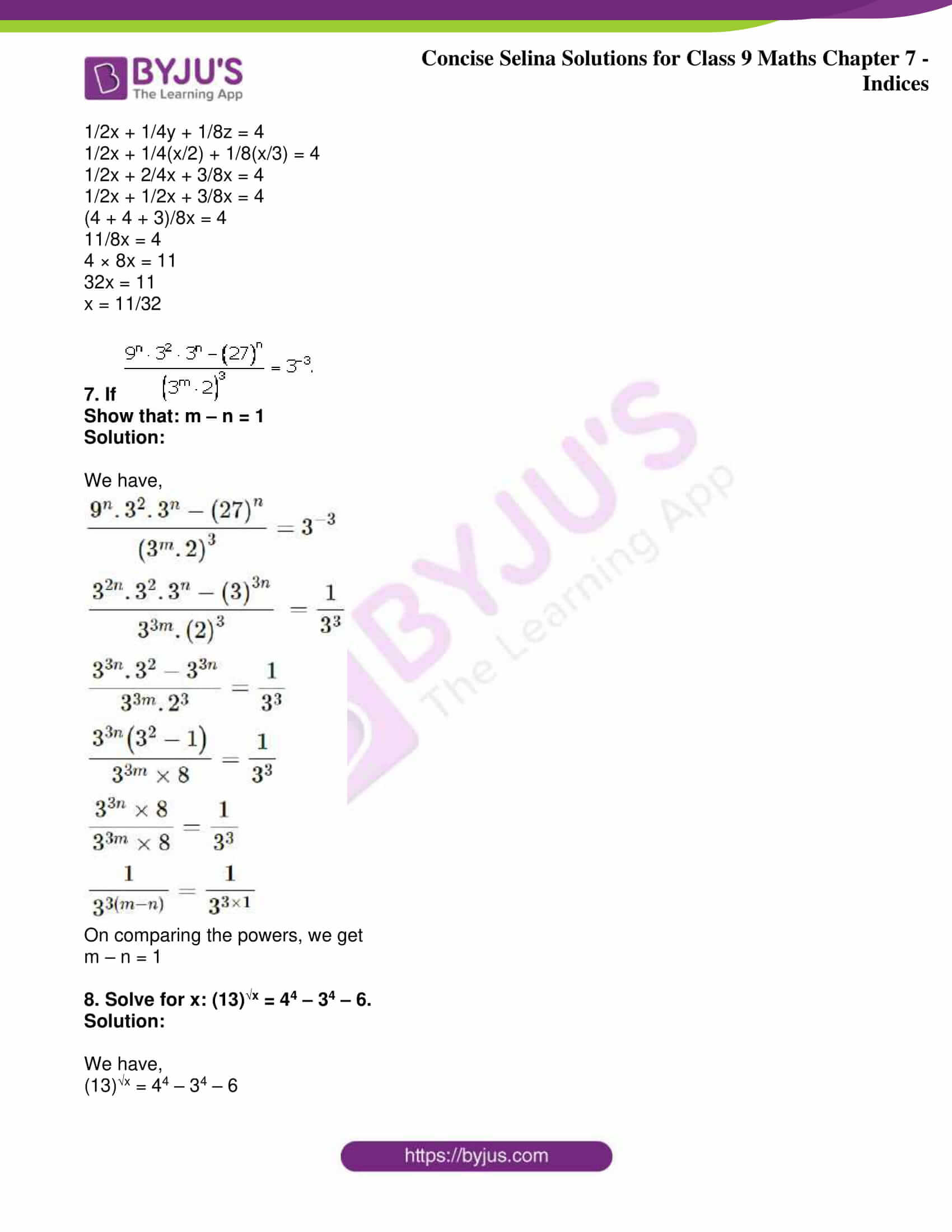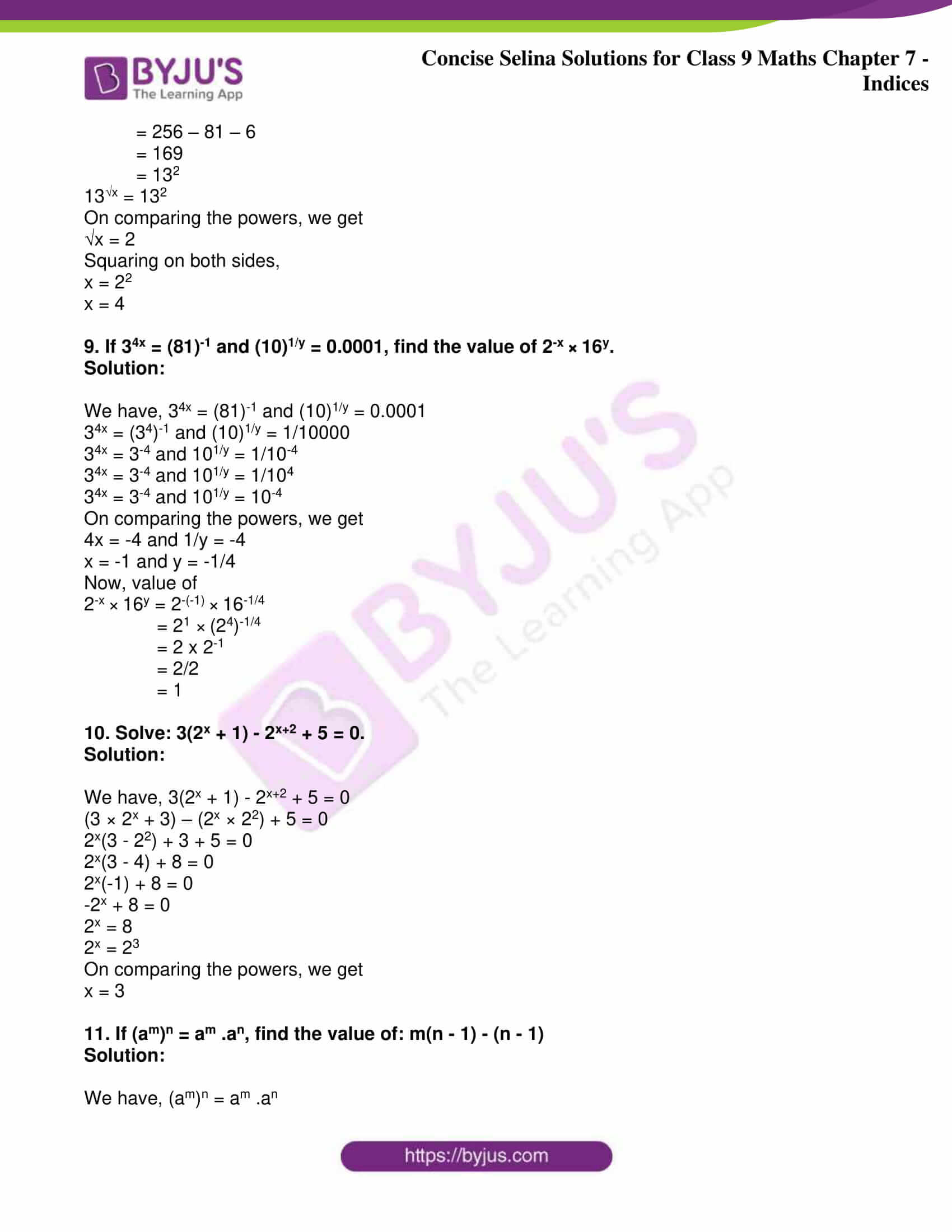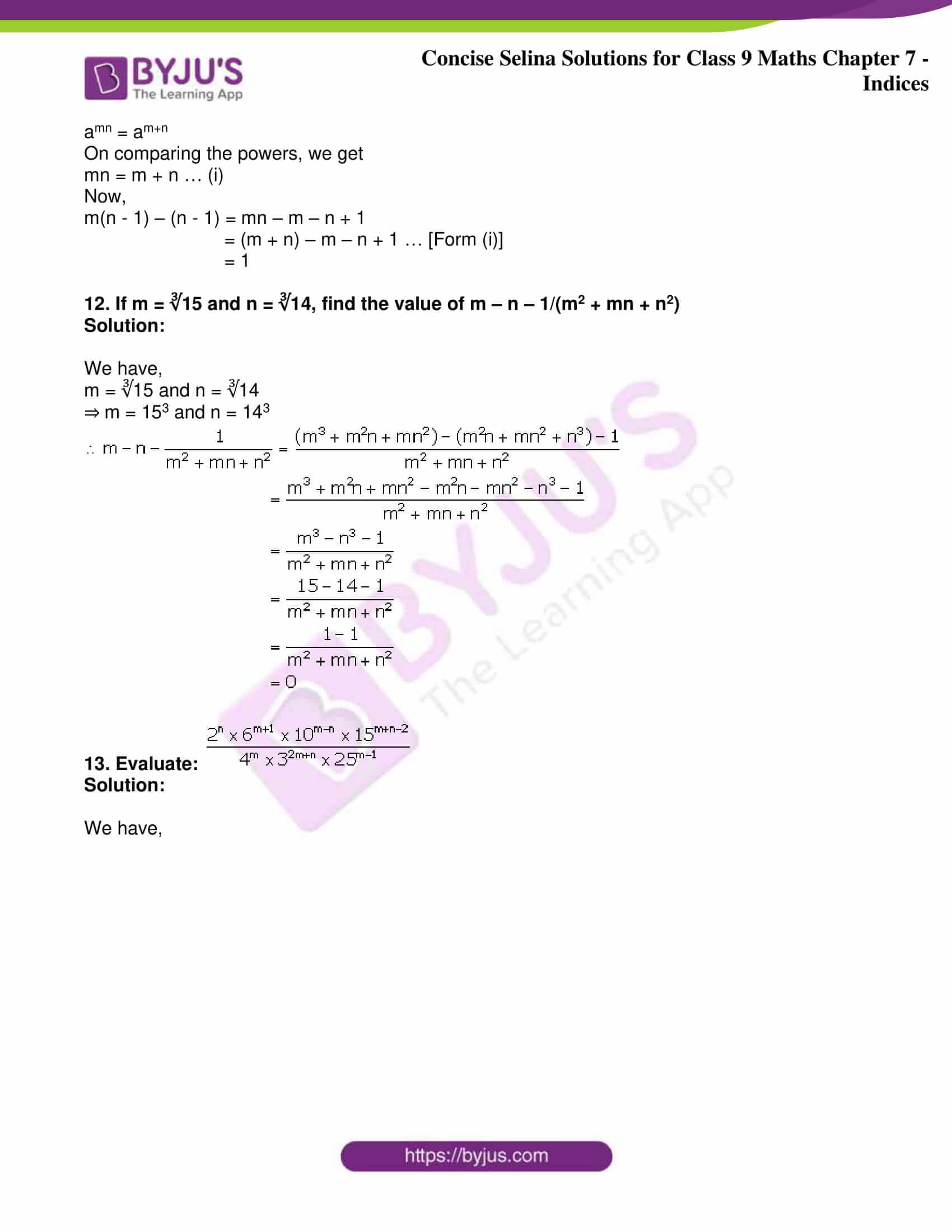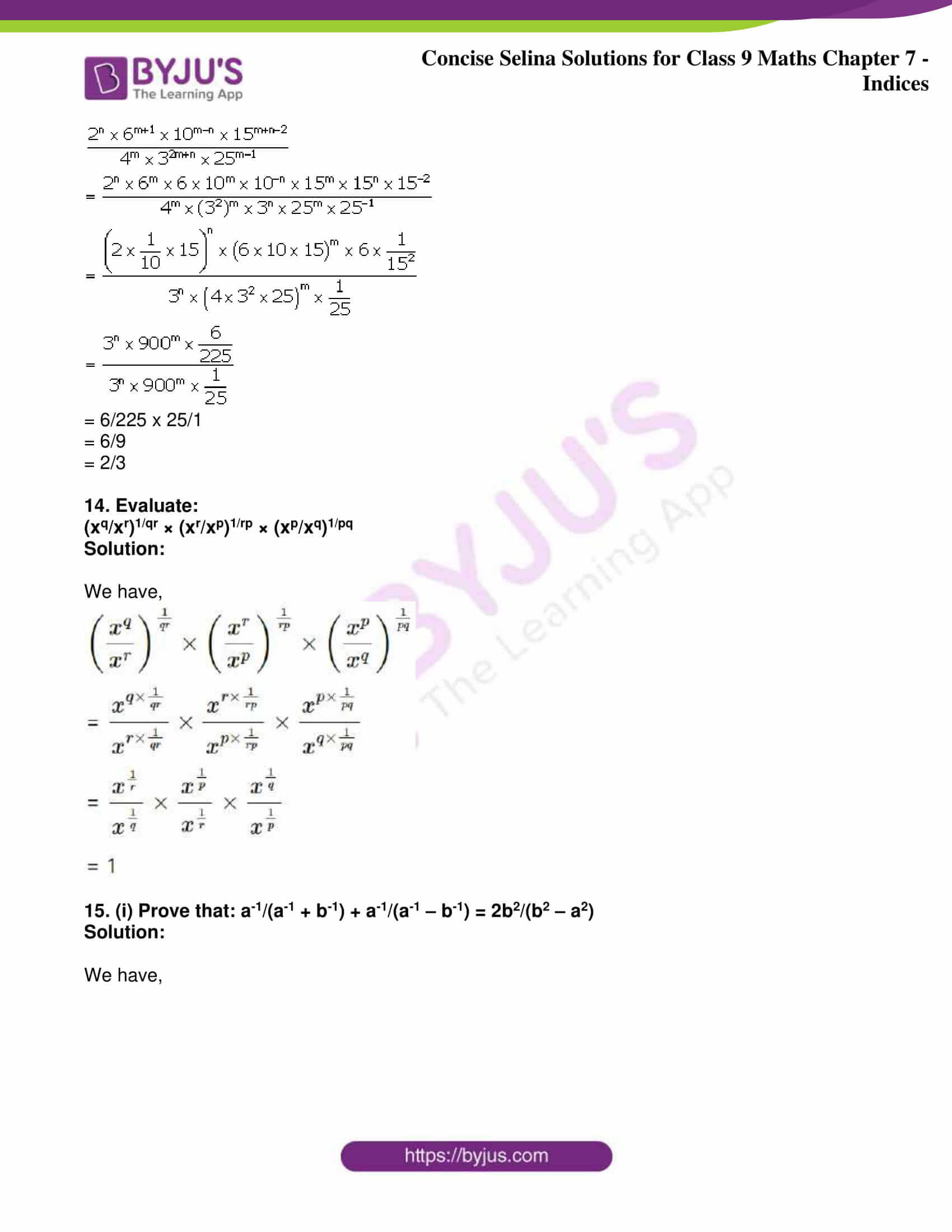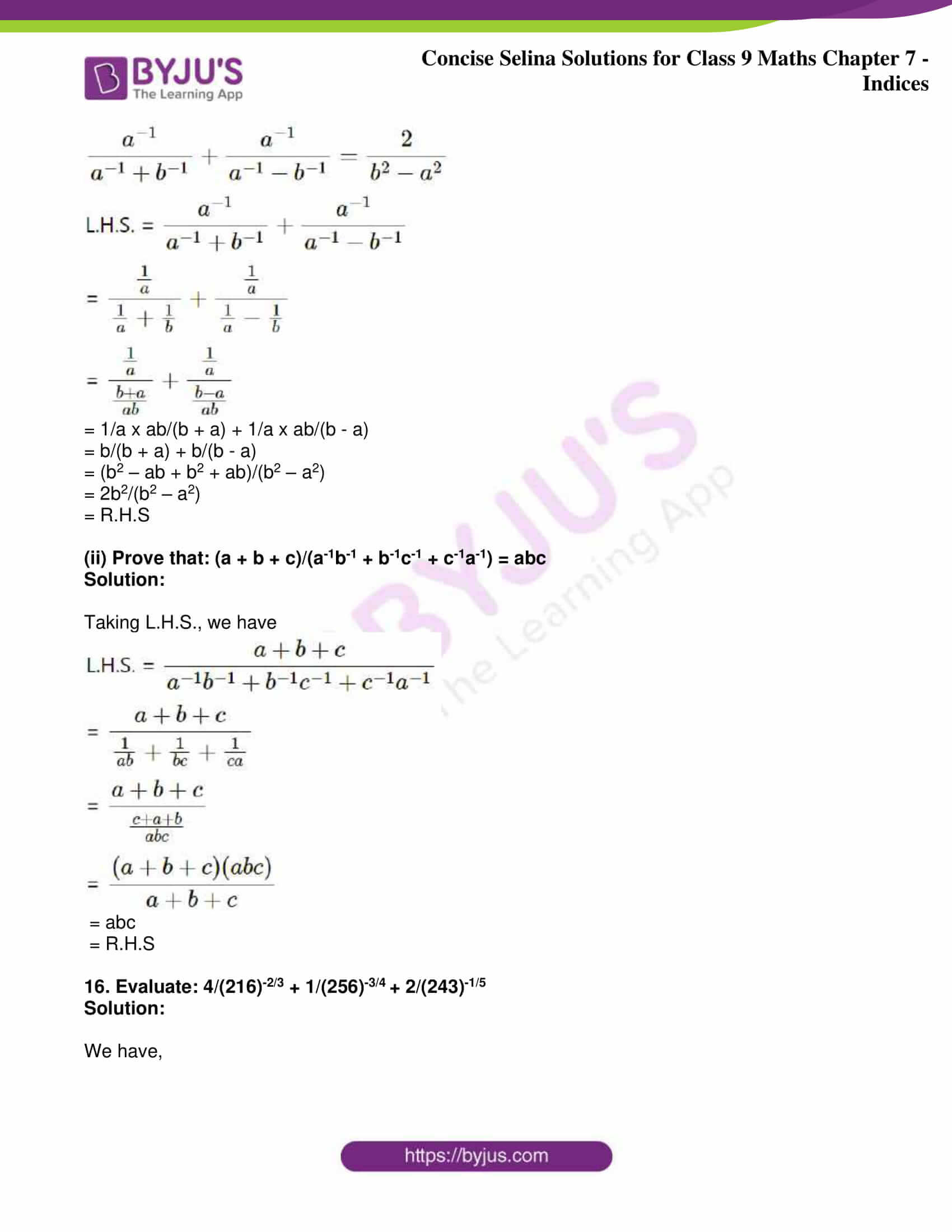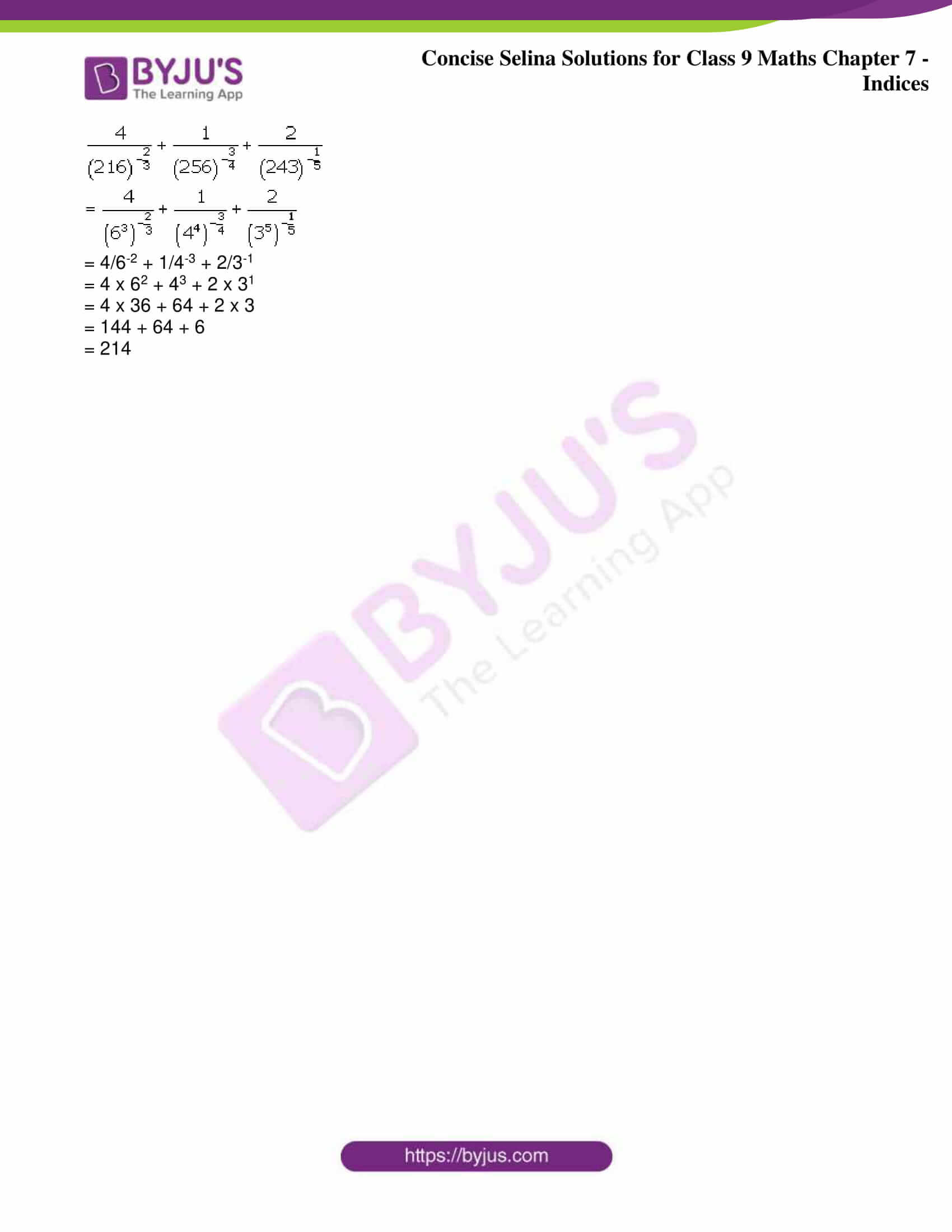Exercise 7(A)

1. Evaluate:

(i) 33 x (243)-2/3 x 9-1/3

(ii) 5-4 x (125)5/3 ÷ (25)-1/2

(iii) (27/125)2/3 x (9/25)-3/2

(iv) 70 x (25)-3/2 – 5-3

(v) (16/81)-3/4 x (49/9)3/2 ÷ (343/216)2/3

Solution:

(i) 33 x (243)-2/3 x 9-1/3 = 33 x (3 x 3 x 3 x 3 x 3)-2/3 x (3 x 3)-1/3

= 33 x (35)-2/3 x (32)-1/3

= 33 x (3)-10/3 x 3-2/3 [As (am)n = amn]

= 33 – 10/3 – 2/3 [am x an x ap = am + n + p]

= 3 (9 – 10 – 2)/3

= 3-3/3

= 3-1

= 1/3

(ii) 5-4 x (125)5/3 ÷ (25)-1/2 = 5-4 x (5 x 5 x 5)5/3 ÷ (5 x 5)-1/2

= 5-4 x (53)5/3 ÷ (52)-1/2

= 5-4 x (53 x 5/3) ÷ (52 x -1/2) [As (am)n = amn]

= 5-4 x 55 ÷ 5-1

= 5-4 x 55 x 5-(-1) [As 1/a-m = a-(-m) = am]

= 5(-4 + 5 + 1) [am x an x ap = am + n + p]

= 52

= 25

(iii) (27/125)2/3 x (9/25)-3/2 = (3 x 3 x 3/5 x 5 x 5)2/3 x (3 x 3/5 x 5)-3/2

= (33/53)2/3 x (32/52)-3/2

= (3/5)3 x 2/3 x (3/5)2 x -3/2 [As (am)n = amn]

= (3/5)2 x (3/5)-3

= (3/5)2 – 3 [am x an = am + n]

= (3/5)-1

= 5/3

(iv) 70 x (25)-3/2 – 5-3 = 1 x (5 x 5)-3/2 – 5-3  [As a0 = 1]

= (52)-3/2 – 5-3

= (5)2 x -3/2 – 5-3 [As (am)n = amn]

= 5-3 – 5-3

= 1/53 – 1/53

= 0

(v) (16/81)-3/4 x (49/9)3/2 ÷ (343/216)2/3

= (2 x 2 x 2 x 2/3 x 3 x 3 x 3)-3/4 x (7 x 7/3 x 3)3/2 ÷ (7 x 7 x 7/6 x 6 x 6)2/3

= (24/34)-3/4 x (72/32)3/2 ÷ (73/63)2/3

= (2/3)4 x -3/4 x (7/3)2 x 3/2 ÷ (7/6)3 x 2/3

= (2/3)-3 x (7/3)3 ÷ (7/6)2 [As (am)n = amn]

= [1/(2/3)3 x (7/3)3]/ (7/6)2 [As a-m = 1/am]

= [(3/2)3 x (7/3)3] x (7/6)-2

= (3/2)3 x (7/3)3 x (6/7)2 [As (a/b)-m = (b/a)m]

= 3/2 x 3/2 x 3/2 x 7/3 x 7/3 x 7/3 x 6/7 x 6/7

= (7 x 3 x 3)/2

= 63/2

= 31.5

2. Simplify:

(i) (8x3 ÷ 125y3)2/3

(ii) (a + b)-1. (a-1 + b-1)

(iii)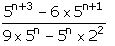(iv) (3x2)-3 × (x9)2/3

Solution:

(i) (8x3 ÷ 125y3)2/3 = (8x3/125y3)2/3

= (2x × 2x × 2x/5y × 5y × 5y)2/3

= (2x3/5y3)2/3

= (2x/5y)3 x 2/3

= (2x/5y)2

= 4x2/25y2

(ii) (a + b)-1. (a-1 + b-1) = 1/(a + b) x (1/a + 1/b)

= 1/(a + b) x (b + a)/ab

= 1/ab

(iii)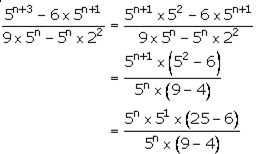= (51 x 19)/5

= 19

(iv) (3x2)-3 × (x9)2/3 = 3-3 × (x2)-3 × (x)9 x 2/3

= 3-3 × (x)2 x -3 × (x)9 x 2/3

= 3-3 × x-6 × x6

= 3-3 x 1

= 1/27

3. Evaluate:

(i) √¼ + (0.01)-1/2 – (27)2/3

(ii) (27/8)2/3 – (1/4)-2 + 50

Solution:

(i) √¼ + (0.01)-1/2 – (27)2/3 = √(½ x ½) + (0.1 x 0.1)-1/2 – (3 x 3 x 3)2/3

= √(½)2 + (0.12) -1/2 – (33)2/3

= ½ + (0.1)2 x -1/2 – (3)3 x 2/3

= ½ + (0.1)-1 – (3)2

= ½ + 1/0.1 – 32

= ½ + 1/(1/10) – 9

= ½ + 10 – 9

= ½ + 1

= 3/2

(ii) (27/8)2/3 – (1/4)-2 + 50 = (3 x 3 x 3/2 x 2 x 2)2/3 – (1/2 x 1/2)-2 + 50

= (33/23)2/3 – (½)2)-2 + 1

= (3/2)3 x 2/3 – (½)-4 + 1

= (3/2)2 – (½)-4 + 1

= (3/2)2 – 24 + 1

= (3 x 3)/(2 x 2) – (2 x 2 x 2 x 2) + 1

= 9/4 – 16 + 1

= (9 – 64 + 4)/4

= -51/4

4. Simplify each of the following and express with positive index:

(i) (3-4/2-8)1/4

(ii) (27-3/9-3)1/5

(iii) (32)-2/5 ÷ (125)-2/3

(iv) [1 – {1 – (1 – n)-1}-1]-1

Solution:

(i) (3-4/2-8)1/4 = (28/34)1/4

= (28)1/4/(34)1/4

= (2)8/4/(3)4/4

= 22/3

= 4/3

(ii) (27-3/9-3)1/5 = (93/273)1/5

= [(3 x 3)3/(3 x 3 x 3)3]1/5

= [(32)3/(33)3]1/5

= [(3)2 x 3/(3)3 x 3]1/5

= [(3)6/(3)9]1/5

= [(3)6-9]1/5

= (3)-3 x 1/5

= (3)-3/5

= 1/33/5

(iii) (32)-2/5 ÷ (125)-2/3 = (32)-2/5/(125)-2/3

= (125)2/3/(32)2/5

= (5 x 5 x 5)2/3/(2 x 2 x 2 x 2 x 2)2/5

= (53)2/3/(25)2/5

= 53 x 2/3/25 x 2/5

= 52/22

= 25/4

(iv) [1 – {1 – (1 – n)-1}-1]-1 = [1 – {1 – 1/(1 – n)}-1]-1

= [1 – {((1 – n) – 1)/(1 – n)}-1]-1

= [1 – {– n/(1 – n)}-1]-1

= [1 – {– (1 – n)/n}]-1

= [1 + (1 – n)/n]-1

= [(n + 1 – n)/n]-1

= [1/n]-1

= n

5. If 2160 = 2a. 3b. 5c, find a, b and c. Hence, calculate the value of 3a x 2-b x 5-c.

Solution:

We have,

2160 = 2a x 3b x 5c

(2 x 2 x 2 x 2) x (3 x 3 x 3) x 5 = 2a x 3b x 5c

24 x 33 x 51 = 2a x 3b x 5c

⇒ 2a x 3b x 5c = 24 x 33 x 51

Comparing the exponents of 2, 3 and 5 on both sides, we get

a = 4, b = 3 and c = 1

Hence, the value

3a x 2-b x 5-c = 34 x 2-3 x 5-1

= (3 x 3 x 3 x 3) x (½ x ½ x ½) x 1/5

= 81 x 1/8 x 1/5

= 81/40

6. If 1960 = 2a. 5b. 7c, calculate the value of 2-a. 7b. 5-c.

Solution:

We have,

1960 = 2a x 5b x 7c

(2 x 2 x 2) x 5 x (7 x 7) = 2a x 5b x 7c

23 x 51 x 72 = 2a x 5b x 7c

⇒ 2a x 5b x 7c = 22 x 51 x 72

Comparing the exponents of 2, 5 and 7 on both sides, we get

a = 3, b = 1 and c = 2

Hence, the value

2-a. 7b. 5-c = 2-3 x 71 x 5-2

= (½ x ½ x ½) x 7 x (1/5 x 1/5)

= 1/8 x 7 x 1/25

= 7/200

7. Simplify:

(i)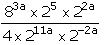(ii)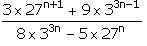Solution:

(i)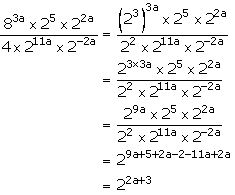(ii)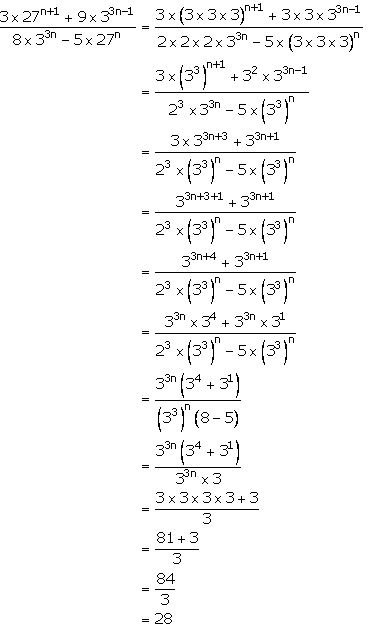8. Show that:

(am/a-n)m-n × (an/a-l)n-l × (al/a-m)l-m = 1

Solution:

Taking the L.H.S., we have

(am/a-n)m-n × (an/a-l)n-l × (al/a-m)l-m

= (am × an)m-n × (an ×al)n-l × (al × am)l-m

= (am+n) m-n × (an+l)n-l × (al+m)l-m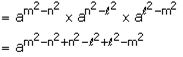= a0

= 1

9. If a = xm+n. xl; b = xn+l. xm and c = xl+m. xn,

Prove that: am-n. bn-l. cl-m = 1

Solution:

We have,

a = xm+n. xl

b = xn+l. xm

c = xl+m. xn

Now,

Considering the L.H.S.,

am-n. bn-l. cl-m

= (xm+n. xl)m-n. (xn+l. xm)n-l. (xl+m. xn)l-m

= [x(m+n)(m-n). xl(m-n)]. [x(n+l)(n-l). xm(n-l)]. [x(l+m)(l-m). xn(l-m)]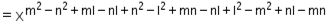= x0

= 1

= R.H.S

– Hence proved.

10. Simplify:

(i)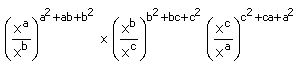(ii)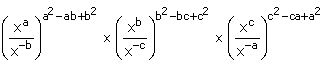Solution:

(i)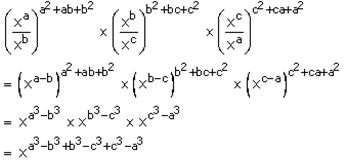= x0

= 1

(ii)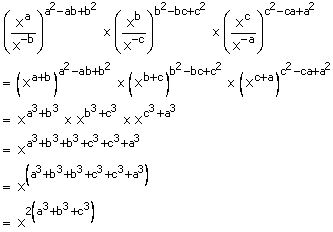Exercise 7(B)

1. Solve for x:

(i) 22x+1 = 8

(ii) 25x-1 = 4 × 23x + 1

(iii) 34x + 1 = (27)x + 1

(iv) (49)x + 4 = 72 × (343)x + 1

Solution:

(i) We have, 22x+1 = 8

⇒22x+1 = 23

Now, if the bases are equal, then the powers must be equal

On comparing the exponents, we get

2x + 1 = 3

2x = 3 – 1

2x = 2

x = 2/2

x = 1

(ii) We have, 25x-1 = 4 × 23x + 1

⇒ 25x-1 = 22 × 23x + 1

25x-1 = 2(3x + 1) + 2

25x-1 = 23x + 3

Now, if the bases are equal, then the powers must be equal

On comparing the exponents, we get

5x – 1 = 3x + 3

5x – 3x = 3 + 1

2x = 4

x = 4/2

x = 2

(iii) We have, 34x + 1 = (27)x + 1

34x + 1 = (33)x + 1

34x + 1 = (3)3x + 3

Now, if the bases are equal, then the powers must be equal

On comparing the exponents, we get

4x + 1 = 3x + 3

4x – 3x = 3 – 1

x = 2

(iv) We have, (49)x + 4 = 72 × (343)x + 1

(7 x 7)x + 4 = 72 × (7 x 7 x 7)x + 1

(72)x + 4 = 72 × (73)x + 1

(7)2x + 8 = (7)3x + 3 + 2

(7)2x + 8 = (7)3x + 5

Now, if the bases are equal, then the powers must be equal

On comparing the exponents, we get

2x + 8 = 3x + 5

3x – 2x = 8 – 5

x = 3

2. Find x, if:

(i) 42x = 1/32

(ii) √(2x+3) = 16

(iii) [√(⅗)]x+1 = 125/27

(iv) [∛(⅔)]x-1 = 27/8

Solution:

(i) We have, 42x = 1/32

(2 × 2)2x = 1/(2 × 2 × 2 × 2 × 2)

(22)2x = 1/25

24x = 2-5

Now, if the bases are equal, then the powers must be equal

So, on comparing the exponents, we get

4x = -5

x = -5/4

(ii) We have, √(2x+3) = 16

(2x+3)1/2 = (2 × 2 × 2 × 2)

2(x+3)/2 = 24

Now, if the bases are equal, then the powers must be equal

So, on comparing the exponents, we get

(x + 3)/2 = 4

x + 3 = 8

x = 8 – 3

x = 5

(iii) We have, [√(⅗)]x+1 = 125/27

[(⅗)1/2]x+1 = (5 × 5 × 5)/(3 × 3 × 3)

(⅗)(x+1)/2 = 53/33

(⅗)(x+1)/2 = (5/3)3

(⅗)(x+1)/2 = (3/5)-3

Now, if the bases are equal, then the powers must be equal

So, on comparing the exponents, we get

(x + 1)/2 = -3

x + 1 = -6

x = -6 – 1

x = -7

(iv) We have, [∛(⅔)]x-1 = 27/8

[(⅔)1/3]x-1 = (3 × 3 × 3)/(2 × 2 × 2)

(⅔)(x-1)/3 = (3/2)3

(⅔)(x-1)/3 = (2/3)-3

Now, if the bases are equal, then the powers must be equal

So, on comparing the exponents, we get

(x – 1)/3 = -3

x – 1 = -9

x = -9 + 1

x = -8

3. Solve:

(i) 4x-2 – 2x+1 = 0

(ii)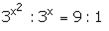Solution:

(i) We have,

4x-2 – 2x+1 = 0

(22)x-2 – 2x+1 = 0

22x-4 – 2x+1 = 0

22x-4 = 2x+1

Now, if the bases are equal, then the powers must be equal

So, on comparing the exponents, we get

2x – 4 = x + 1

2x – x = 4 + 1

x = 5

(ii) We have,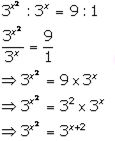Now, if the bases are equal, then the powers must be equal

So, on comparing the exponents, we get

x2 = x+ 2

x2 – x – 2 = 0

On factorization, we get

x2 – 2x + x – 2 = 0

x(x – 2 + 1(x – 2) = 0

(x + 1)(x – 2) = 0

So, either (x + 1) = 0 or (x – 2) = 0

Thus, x = -1 or 2

4. Solve:

(i) 8 × 22x + 4 × 2x+1 = 1 + 2x

(ii) 22x + 2x+2 – 4 × 23 = 0

(iii) (√3)x-3 = (∜3)x+1

Solution:

(i) We have, 8 × 22x + 4 × 2x+1 = 1 + 2x

8 × (2x)2 + 4 × (2x) × 21 = 1 + 2x

Let us substitute 2x = t

Then,

8 × t2 + 4 × t × 2 = 1 + t

8t2 + 8t = 1 + t

8t2 + 8t – t – 1 = 0

8t2 + 7t – 1 = 0

8t2 + 8t – t – 1 = 0

8t(t + 1) – 1(t + 1) = 0

(8t – 1)(t + 1) = 0

So, either 8t – 1 = 0 or t + 1 = 0

Thus, t = 1/8 or -1

Now, we have

2x = t

So,

2x = 1/8 or 2x = -1

The equation, 2x = -1 is not possible

Hence, for 2x = 1/8

2x = 1/(2 × 2 × 2)

2x = 1/23

2x = 2-3

Now, if the bases are equal, then the powers must be equal

So, on comparing the exponents, we get

x = -3

(ii) We have,

22x + 2x+2 – 4 × 23 = 0

22x + 2x+2 – 22 × 23 = 0

(2x)2 + 2x.22 – 23+2 = 0

(2x)2 + 2x.22 – 25 = 0

Now, let’s assume 2x = t

So, the above equation becomes

(t)2 + t.22 – 25 = 0

t2 + 4t – 32 = 0

On factorization, we have

t2 + 8t – 4t – 32 = 0

t(t + 8) – 4(t + 8) = 0

(t – 4)(t + 8) = 0

So, either (t – 4) = 0 or (t + 8) = 0

Thus, t = 4 or -8

Now, we have t = 2x

So,

2x = 4 or 2x = -8

The equation, 2x = -8 is not possible

Hence, for

2x = 4

2x = 22

On comparing the exponents, we get

x = 2

(iii) (√3)x-3 = (∜3)x+1

(31/2)x-3 = (31/4)x+1

3(x-3)/2 = 3(x+1)/4

Now, if the bases are equal, then the powers must be equal

So, on comparing the exponents, we get

(x – 3)/2 = (x + 1)/4

2(x – 3) = (x + 1)

2x – 6 = x + 1

2x – x = 6 + 1

x = 7

5. Find the values of m and n if:

42m = (∛16)-6/n = (√8)2

Solution:

We have, 42m = (∛16)-6/n = (√8)2

Now, considering

42m = (√8)2

(22)2m = (81/2)2

24m = 81/2 x 2

24m = 8

⇒ 24m = 23

Now, if the bases are equal, then the powers must be equal

So, on comparing the exponents, we get

4m = 3

m = ¾

Now, from the given considering

(∛16)-6/n = (√8)2

(161/3)-6/n = (81/2)2

(16)1/3 x -6/n = 81/2 x 2

(16)-2/n = 8

(2 x 2 x 2 x 2)-2/n = (2 x 2 x 2)

(2)4 x -2/n = 23

(2)-8/n = 23

Now, if the bases are equal, then the powers must be equal

So, on comparing the exponents, we get

-8/n = 3

n = -8/3

Therefore, the value of m and n are ¾ and -8/3

6. Solve x and y if:

(√32)x ÷ 2y+1 = 1 and 8y – 164 – x/2 = 0

Solution:

Consider the equation, (√32)x ÷ 2y+1 = 1

(√(2 × 2 × 2 × 2 × 2))x ÷ 2y+1 = 1

(√25)x ÷ 2y+1 = 1

(25)1/2 × x ÷ 2y+1 = 1

25x/2 ÷ 2y+1 = 1

(25x/2)/(2y+1) = 1

25x/2 – (y+1) = 20

Now, if the bases are equal, then the powers must be equal

So, on comparing the exponents, we get

5x/2 – (y+1) = 0

5x/2 – y – 1 = 0

5x – 2y – 2 = 0 … (i)

Next, let’s consider 8y – 164 – x/2 = 0

(2 × 2 × 2)y – (2 × 2 × 2 × 2)4 – x/2 = 0

(23)y – (24)4 – x/2 = 0

23y – 216 – 2x = 0

23y = 216 – 2x

Now, if the bases are equal, then the powers must be equal

So, on comparing the exponents, we get

3y = 16 – 2x

2x + 3y – 16 = 0 … (ii)

On solving equations (i) and (ii),

By manipulating by (i) × 3 + (ii) × 2, we have

15x – 6y – 6 = 0

4x + 6y – 32 = 0

———————–

19x – 38 = 0

x = 38/19

x = 2

Now, substituting the value of x in (i)

5(2) – 2y – 2 = 0

10 – 2y – 2 = 0

8 = 2y

y = 8/2

y = 4

Therefore, the values of x and y are 2 and 4 respectively

7. Prove that:

(i) (xa/xb)a+b-c. (xb/xc)b+c-a. (xc/xa)c+a-b = 1

(ii) xa(b-c)/xb(a-c) ÷ (xb/xa)c = 1

Solution:

(i) Taking L.H.S, we have

(xa/xb)a+b-c. (xb/xc)b+c-a. (xc/xa)c+a-b

= (xa/xb)a+b-c. (xb/xc)b+c-a. (xc/xa)c+a-b

= x(a-b)(a+b-c). x(b-c)(b+c-a). x(c-a)(c+a-b)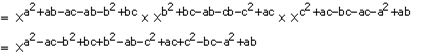= x0

= 1

= R.H.S

(ii) Taking L.H.S, we have

xa(b-c)/xb(a-c) ÷ (xb/xa)c

= xa(b-c) – b(a-c) ÷ xc(b-a)

= xa(b-c) – b(a-c)/xc(b-a)

= xa(b-c) – b(a-c) – c(b-a)

= xab-ac-ba+bc-cb+ac

= x0

= 1

= R.H.S

8. If ax = b, by = c and cz = a, prove that: xyz = 1.

Solution:

We have, ax = b, by = c and cz = a

Now, considering

ax = b

On raising to the power yz on both sides, we get

(ax)yz = (b)yz

(a)xyz = (by)z

(a)xyz = (c)z [As, by = c]

axyz = a

axyz = a1 [As, cz = a]

Now, if the bases are equal, then the powers must be equal

On comparing the exponents, we get

Hence, xyz = 1

9. If ax = by = cz and b2 = ac, prove that: y = 2az/(x + z).

Solution:

Let’s assume ax = by = cz = k

So,

a = k1/x; b = k1/y and c = k1/z

Now,

It’s given that b2 = ac

⇒ (k1/y)2 = (k1/x) × (k1/z)

(k2/y) = k1/x + 1/z

k2/y = k(z+x)/xz

Now, if the bases are equal, then the powers must be equal

On comparing the exponents, we get

2/y = (z + x)/xz

2xz = y(z + x)

Hence,

y = 2xz/(x + z)

10. If 5-p = 4-q = 20r, show that: 1/p + 1/q + 1/r = 0.

Solution:

Let’s assume 5-p = 4-q = 20r = k

Then, as

5-p = k ⇒ 5 = k-1/p

4-q = k ⇒ 4 = k-1/q

20r = k ⇒ 20 = k1/r

Now, we know

5 x 4 = 20

(k-1/p) x (k-1/q) = k1/r

k-1/p – 1/q = k1/r

Now, if the bases are equal, then the powers must be equal

On comparing the exponents, we get

-1/p – 1/q = 1/r

Hence,

1/p + 1/q + 1/r = 0

11. If m ≠ n and (m + n)-1 (m-1 + n-1) = mxny, show that: x + y + 2 = 0

Solution:

Given equation,

(m + n)-1 (m-1 + n-1) = mxny

1/(m + n) × (1/m + 1/n) = mxny

1/(m + n) × (m + n)/mn = mxny

1/mn = mxny

m-1n-1 = mxny

Now, if the bases are equal, then the powers must be equal

On comparing the exponents, we get

x = -1 and y = -1

Substituting the values of x and y in the equation x + y + 2 = 0, we have

(-1) + (-1) + 2 = 0

0 = 0

L.H.S = R.H.S

Therefore, x + y + 2 = 0

12. If 5x + 1 = 25x – 2, find the value of 3x – 3 × 23 – x

Solution:

We have, 5x + 1 = 25x – 2

5x + 1 = (52)x – 2

5x + 1 = 52x – 4

Now, if the bases are equal, then the powers must be equal

On comparing the exponents, we get

x + 1 = 2x – 4

2x – x = 4 + 1

x = 5

Hence, the value of

3x – 3 × 23 – x = 35 – 3 × 23 – 5

= 32 × 2-2

= 9 × ¼

= 9/4

13. If 4x + 3 = 112 + 8 × 4x, find the value of (18x)3x.

Solution:

We have,

4x + 3 = 112 + 8 × 4x

4x .43 = 112 + 8 × 4x

Let’s assume 4x = t

Then,

t .43 = 112 + 8 × t

64t = 112 + 8t

64t – 8t = 112

56t = 112

t = 112/56

t = 2

But we have taken 4x = t

So, 4x = 2

(22)x = 21

22x = 21

Now, if the bases are equal, then the powers must be equal

On comparing the exponents, we get

2x = 1

x = ½

Now, the value of (18x)3x will be

= (18 × ½)3 × 1/2

= (9)3/2

= (32)3/2

= 33

= 27

14. Solve for x:

(i) 4x-1 × (0.5)3 – 2x = (1/8)-x

Solution:

We have,

4x-1 × (0.5)3 – 2x = (1/8)-x

(22)x-1 × (1/2)3 – 2x = (1/23)-x

(2)2x-1 × (2)-(3 – 2x) = (2-3)-x

(2)2x-2 × (2)2x-3 = (2)3x

2(2x-2) + (2x-3) = (2)3x

24x-5 = 23x

Now, if the bases are equal, then the powers must be equal

On comparing the exponents, we get

4x – 5 = 3x

4x – 3x = 5

x = 5

(ii) (a3x + 5)2 × (ax)4 = a8x + 12

Solution:

We have,

(a3x + 5)2 × (ax)4 = a8x + 12

a6x + 10 × a4x = a8x + 12

a6x + 10 + 4x = a8x + 12

a10x + 10 = a8x + 12

Now, if the bases are equal, then the powers must be equal

On comparing the exponents, we get

10x + 10 = 8x + 12

10x – 8x = 12 – 10

2x = 2

x = 1

(iii) (81)3/4 – (1/32)-2/5 + x(1/2)-1 × 20 = 27

Solution:

We have,

(81)3/4 – (1/32)-2/5 + x(1/2)-1 × 20 = 27

(34)3/4 – (1/25)-2/5 + x(1/2)-1 × 20 = 27

(34)3/4 – (2-5)-2/5 + x(2-1)-1 × 20 = 27

33 – 22 + 2x × 1 = 27

27 – 4 + 2x = 27

2x + 23 = 27

2x = 27 – 23

2x = 4

x = 4/2

x = 2

(iv) 23x + 3 = 23x + 1 + 48

Solution:

We have,

23x + 3 = 23x + 1 + 48

23x + 3 – 23x + 1 = 48

23x(23 – 21) = 48

23x(8 – 2) = 48

23x × 6 = 48

23x = 48/6

23x = 8

23x = 23

Now, if the bases are equal, then the powers must be equal

On comparing the exponents, we get

3x = 3

x = 1

(v) 3(2x + 1) – 2x+2 + 5 = 0

Solution:

We have,

3(2x + 1) – 2x+2 + 5 = 0

3 × 2x + 3 – 2x.22 + 5 = 0

2x(3 – 22) + 5 + 3 = 0

2x(3 – 4) + 8 = 0

-2x + 8 = 0

2x = 8

2x = 23

Now, if the bases are equal, then the powers must be equal

On comparing the exponents, we get

x = 3

(vi) 9x+2 = 720 + 9x

Solution:

We have,

9x+2 = 720 + 9x

9x+2 – 9x = 720

9x (92 – 1) = 720

9x (81 – 1) = 720

9x (80) = 720

9x = 9

9x = 91

Therefore, x = 1

Exercise 7(C)

1. Evaluate:

(i) 95/2 – 3 x 80 – (1/81)-1/2

(ii) (64)2/3 – ∛125 – 1/2-5 + (27)-2/3 x (25/9)-1/2

(iii) [(-2/3)-2]3 x (1/3)-4 x 3-1 x 1/6

Solution:

(i) We have, 95/2 – 3 x 80 – (1/81)-1/2

= (32)5/2 – 3 x 1 – (1/92)-1/2

= 32 x 5/2 – 3 – (1/9)-2 x ½

= 35 – 3 – (1/9)-1

= (3 x 3 x 3 x 3 x 3) – 3 – (9-1)-1

= 243 – 3 – 9

= 231

(ii) We have, (64)2/3 – ∛125 – 1/2-5 + (27)-2/3 x (25/9)-1/2

= (4 x 4 x 4)2/3 – (5 x 5 x 5)1/3 – 1/2-5 + (3 x 3 x 3)-2/3 x (5 x 5/3 x 3)-1/2

= (43)2/3 – (53)1/3 – (2-1)-5 + (33)-2/3 x (52/32)-1/2

= (4)3 x 2/3 – (5)3 x 1/3 – (2)-1 x -5 + (3)3 x -2/3 x (5/3)2 x -1/2

= (4)2 – 5 – 25 + 3-2 x (5/3)-1

= 16 – 5 – 32 + 1/9 x 3/5

= -21 + 1/15

= (-315 + 1)/15

= -314/15

(iii) We have, [(-2/3)-2]3 x (1/3)-4 x 3-1 x 1/6

= (-2/3)-6 x (3-1)-4 x 3-1 x ½ x 1/3

= (-3/2)6 x 34 x 3-1 x 2-1 x 3-1

= (-1)6 x (36 x 34 x 3-1 x 3-1) x (2-6 x 2-1)

= 1 x 36+4-1-1 x 2-6-1

= 38 x 2-7

= 38/27

2. Simplify: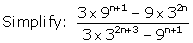Solution:

We have,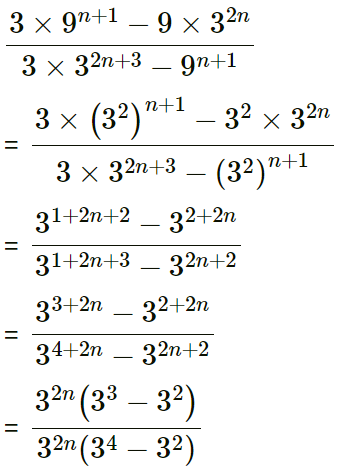= (27 – 9)/(81 – 9)

= 18/72

= 1/4

3. Solve: 3x-1 × 52y-3 = 225.

Solution:

Given, 3x-1× 52y-3 = 225

3x-1 × 52y-3 = 9 x 25

3x-1 × 52y-3 = 32 x 52

Now, if the bases are equal, then the powers must be equal

On comparing the exponents, we get

x – 1 = 2 and 2y – 3 = 2

x = 2 + 1 and 2y = 2 + 3

x = 3 and 2y = 5

x = 3 and y = 5/2 = 2.5

4. If [(a-1b2)/(a2b-4)]7 ÷ [(a3b-5)/(a-2b3)] = ax.by, find x + y.

Solution:

We have,(b42/a21) ÷ (b40/a25) = ax. by

(b42/a21) × (a25/b40) = ax. by

b42 – 40 × a25 – 21 = ax. by

a4 × b2 = ax. by

Now, if the bases are equal, then the powers must be equal

On comparing the exponents, we get

x = 4 and y = 2

x + y = 4 + 2 = 6

5. If 3x+1 = 9x-3, find the value of 21+x.

Solution:

We have,

3x+1 = 9x-3

3x × 31 = (32)x – 3

3x × 31 = (3)2x – 6

3x = (3)2x – 6/3

3x = (3)2x – 6 × 3-1

3x = (3)2x – 6 – 1

3x = 32x – 7

Now, if the bases are equal, then the powers must be equal

On comparing the exponents, we get

x = 2x – 7

x = 7

Now,

21+x = 21+7 = 28 = 256

6. If 2x = 4y = 8z and 1/2x + 1/4y + 1/8z = 4, find the value of x.

Solution:

Given, 2x = 4y = 8z

2x = (22)y = (23)z

2x = 22y = 23z

On comparing the powers, we get

x = 2y = 3z

y = x/2 and z = x/3

Now,

1/2x + 1/4y + 1/8z = 4

1/2x + 1/4(x/2) + 1/8(x/3) = 4

1/2x + 2/4x + 3/8x = 4

1/2x + 1/2x + 3/8x = 4

(4 + 4 + 3)/8x = 4

11/8x = 4

4 × 8x = 11

32x = 11

x = 11/32

7. IfShow that: m – n = 1

Solution:

We have,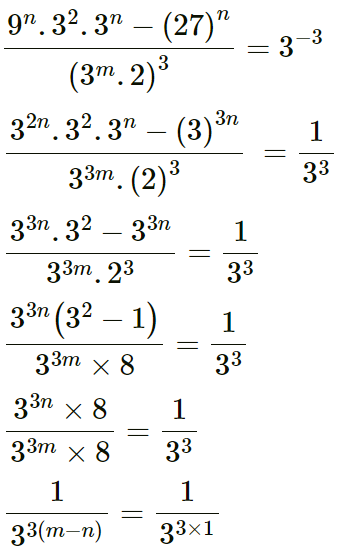On comparing the powers, we get

m – n = 1

8. Solve for x: (13)√x = 44 – 34 – 6.

Solution:

We have,

(13)√x = 44 – 34 – 6

= 256 – 81 – 6

= 169

= 132

13√x = 132

On comparing the powers, we get

√x = 2

Squaring on both sides,

x = 22

x = 4

9. If 34x = (81)-1 and (10)1/y = 0.0001, find the value of 2-x × 16y.

Solution:

We have, 34x = (81)-1 and (10)1/y = 0.0001

34x = (34)-1 and (10)1/y = 1/10000

34x = 3-4 and 101/y = 1/10-4

34x = 3-4 and 101/y = 1/104

34x = 3-4 and 101/y = 10-4

On comparing the powers, we get

4x = -4 and 1/y = -4

x = -1 and y = -1/4

Now, value of

2-x × 16y = 2-(-1) × 16-1/4

= 21 × (24)-1/4

= 2 x 2-1

= 2/2

= 1

10. Solve: 3(2x + 1) – 2x+2 + 5 = 0.

Solution:

We have, 3(2x + 1) – 2x+2 + 5 = 0

(3 × 2x + 3) – (2x × 22) + 5 = 0

2x(3 – 22) + 3 + 5 = 0

2x(3 – 4) + 8 = 0

2x(-1) + 8 = 0

-2x + 8 = 0

2x = 8

2x = 23

On comparing the powers, we get

x = 3

11. If (am)n = am .an, find the value of: m(n – 1) – (n – 1)

Solution:

We have, (am)n = am .an

amn = am+n

On comparing the powers, we get

mn = m + n … (i)

Now,

m(n – 1) – (n – 1) = mn – m – n + 1

= (m + n) – m – n + 1 … [Form (i)]

= 1

12. If m = ∛15 and n = ∛14, find the value of m – n – 1/(m2 + mn + n2)

Solution:

We have,

m = ∛15 and n = ∛14

⇒ m = 153 and n = 14313. Evaluate: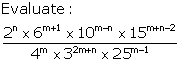Solution:

We have,= 6/225 x 25/1

= 6/9

= 2/3

14. Evaluate:

(xq/xr)1/qr × (xr/xp)1/rp × (xp/xq)1/pq

Solution:

We have,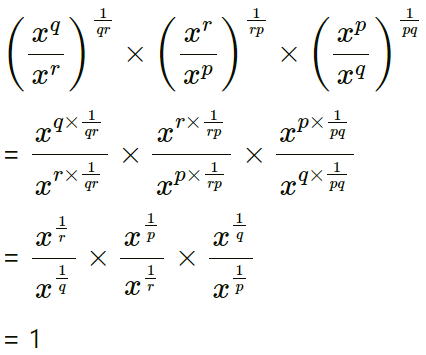15. (i) Prove that: a-1/(a-1 + b-1) + a-1/(a-1 – b-1) = 2b2/(b2 – a2)

Solution:

We have,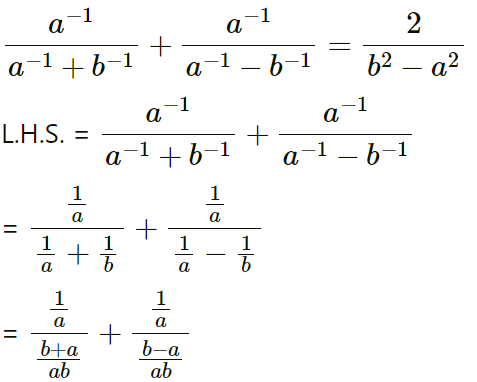= 1/a x ab/(b + a) + 1/a x ab/(b – a)

= b/(b + a) + b/(b – a)

= (b2 – ab + b2 + ab)/(b2 – a2)

= 2b2/(b2 – a2)

= R.H.S

(ii) Prove that: (a + b + c)/(a-1b-1 + b-1c-1 + c-1a-1) = abc

Solution:

Taking L.H.S., we have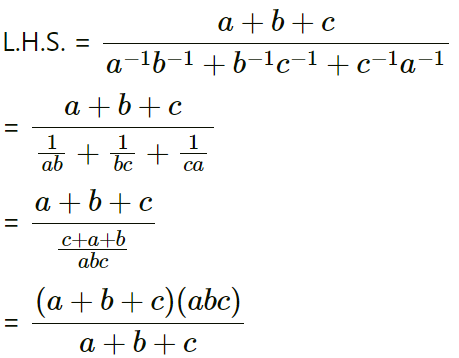= abc

= R.H.S

16. Evaluate: 4/(216)-2/3 + 1/(256)-3/4 + 2/(243)-1/5

Solution:

We have,= 4/6-2 + 1/4-3 + 2/3-1

= 4 x 62 + 43 + 2 x 31

= 4 x 36 + 64 + 2 x 3

= 144 + 64 + 6

= 214

## Selina Solutions for Class 9 Maths Chapter 7- Indices [Exponents]

The Chapter 7, Indices [Exponents], contains 3 exercises and the Solutions given here contains the answers for all the questions present in these exercises. Let us have a look at some of the topics that are being discussed in this chapter.

7.1 Introduction

7.2 Laws of Indices [Exponents]

7.3 Handling positive, fractional, negative and zero indices

7.4 Simplification of expressions

7.5 Solving exponential equations

## Selina Solutions for Class 9 Maths Chapter 7- Indices [Exponents]

The Chapter 7 of class 9 takes the students to a new topic Indices[exponents]. If m is a positive integer, then axaxaxa—- upto m terms, is written as am; where ‘a’ is called the base and m is called the power (or exponent or index). Read and learn the Chapter 7 of Selina textbook to familiarize with the concepts related to exponents or indices. Learn the Selina Solutions for Class 9 effectively to score high in the examination.